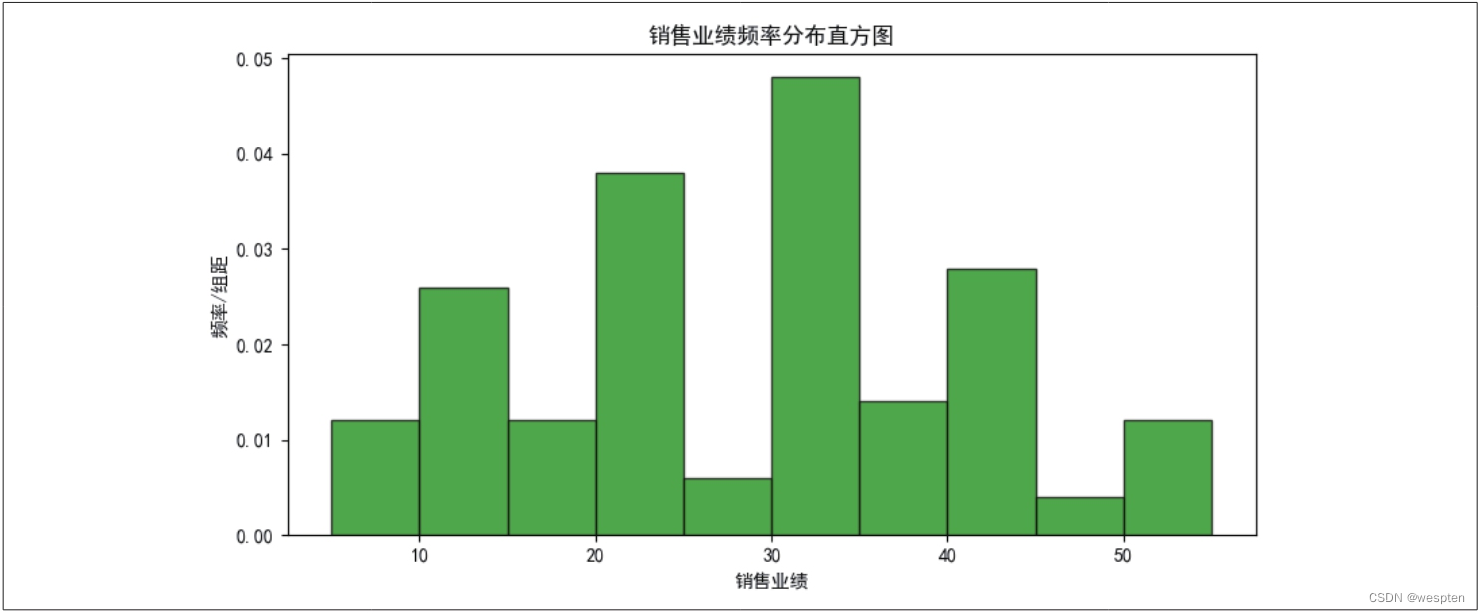## 一、Python环境搭建与配置

Python作为一门优秀的编程语言，受到很多程序员和编程爱好者的青睐。近年来，Python还在办公领域大展拳脚，许多白领纷纷加入了学习Python的行列。这是因为Python在数据的采集、处理、分析与可视化方面有着独特的优势，能够帮助职场人士从容应对大数据时代的挑战。

### 1、Python编程环境的搭建

Anaconda是Python的一个发行版本，安装好了Anaconda就相当于安装好了Python，并且它里面还集成了很多大数据分析与科学计算的第三方模块，如NumPy、pandas、Matplotlib等。

PyCharm则是一款Python代码编辑器，它比Anaconda自带的两款编辑器Spyder和Jupyter Notebook更好用。

1. 安装与配置Anaconda

https://www.anaconda.com/products/individual，进入Anaconda的下载页面，向下滚动页面，在“Anaconda Installers”栏目中可看到与不同类型的计算机操作系统对应的安装包，这里选择适用于64位Windows系统的Python 3.8版本，如下图所示。2. 安装与配置PyCharm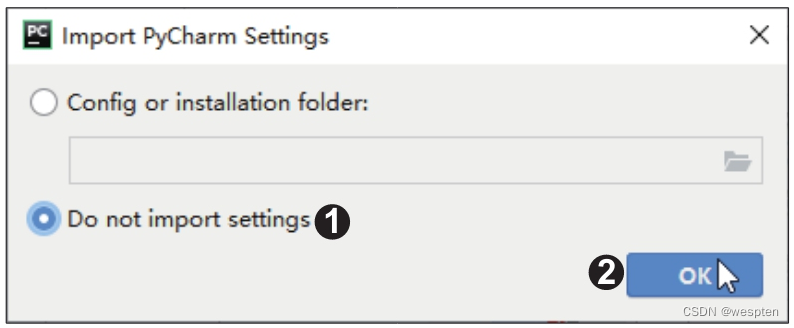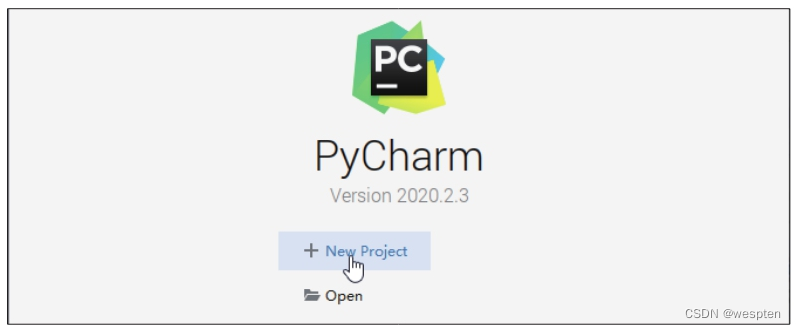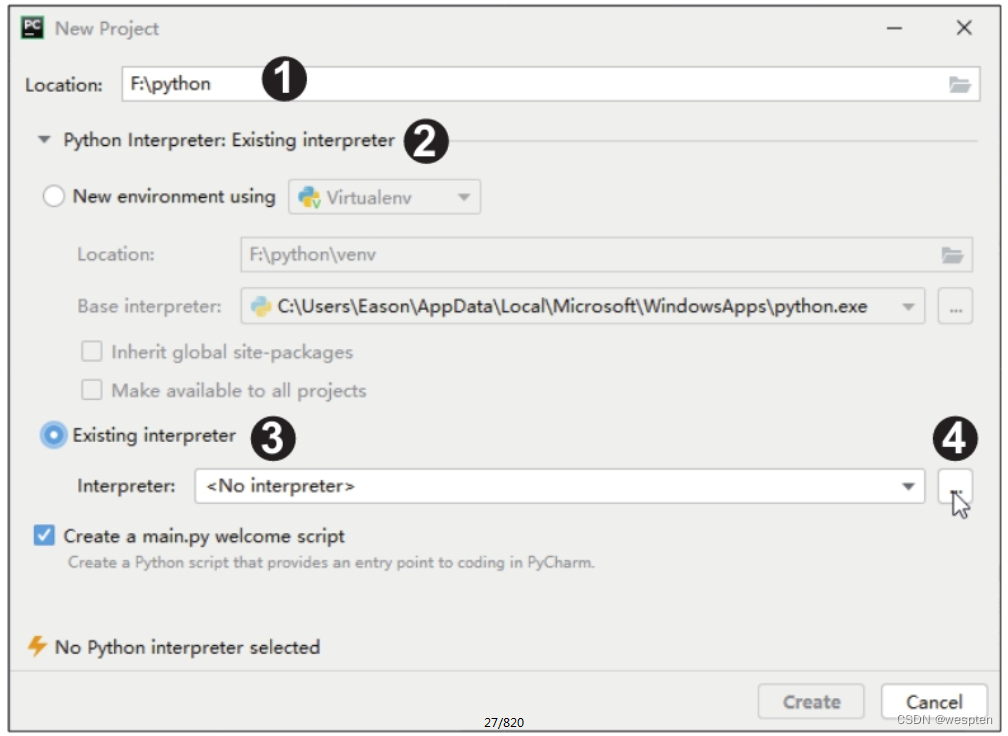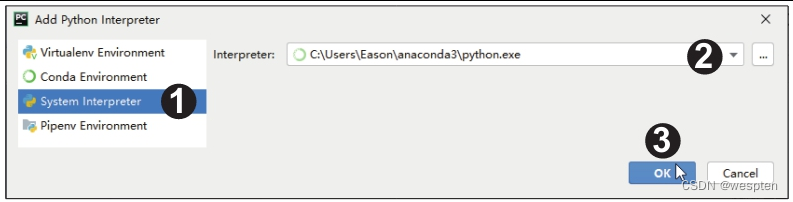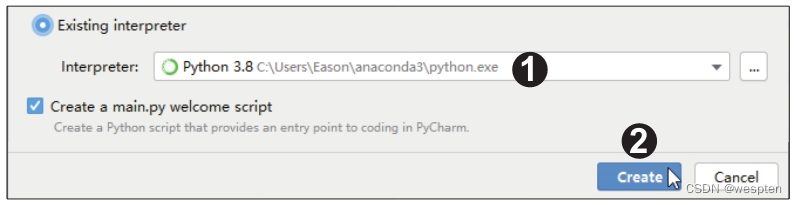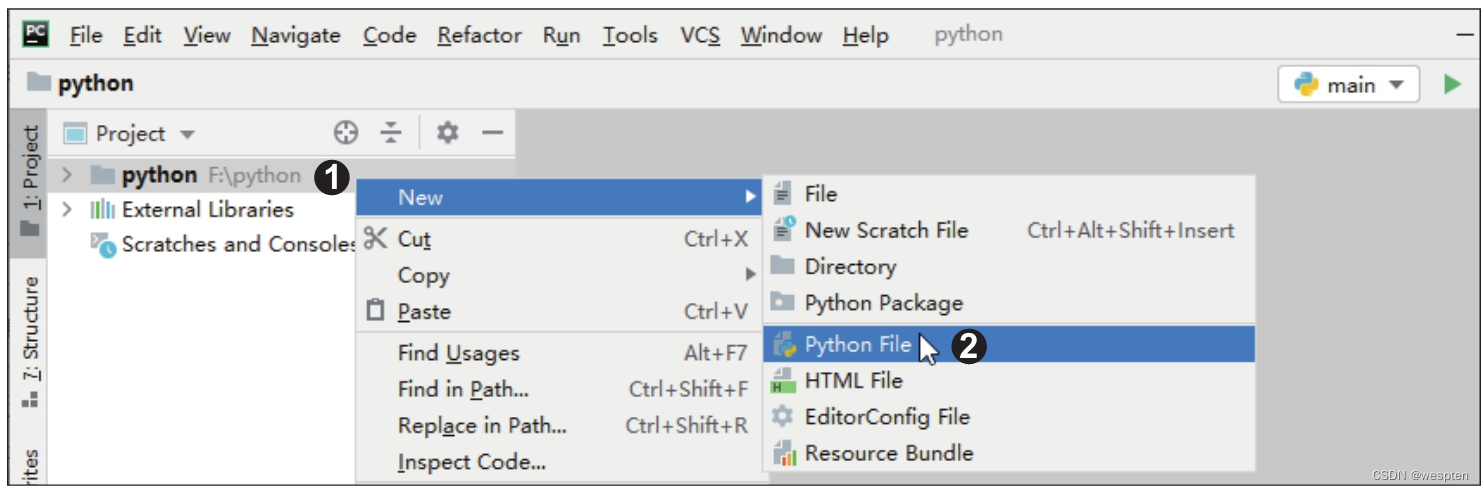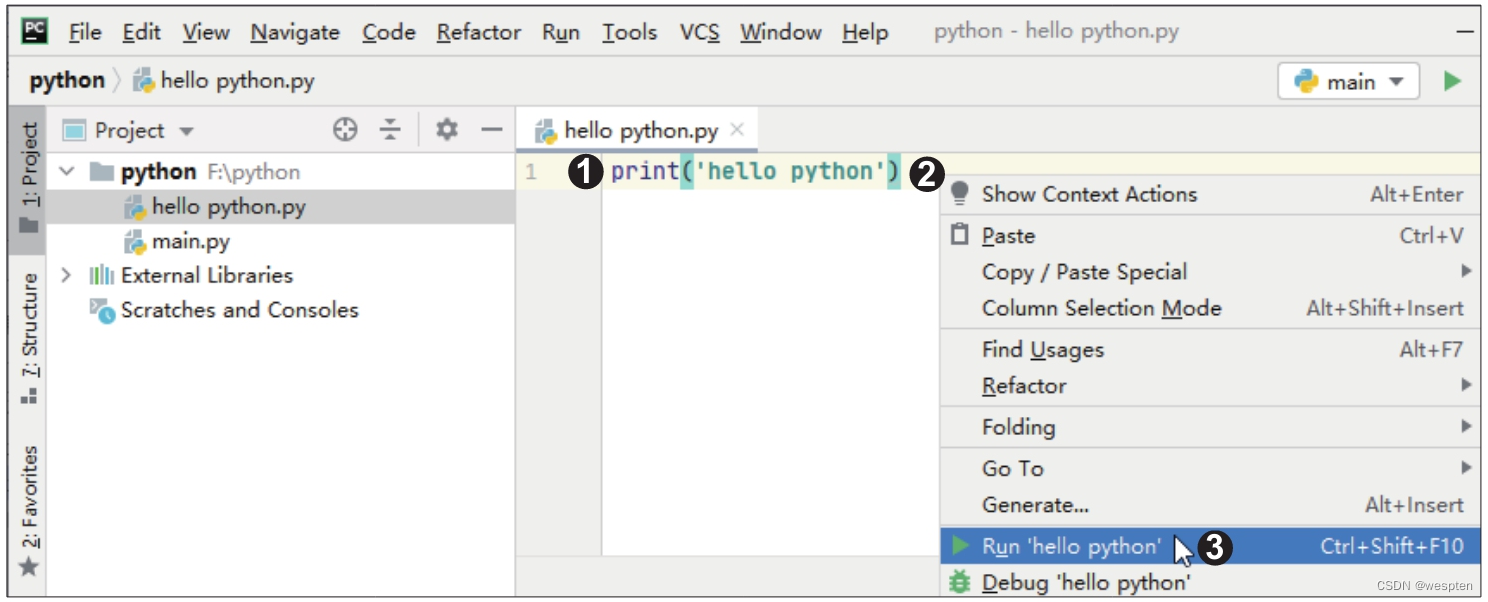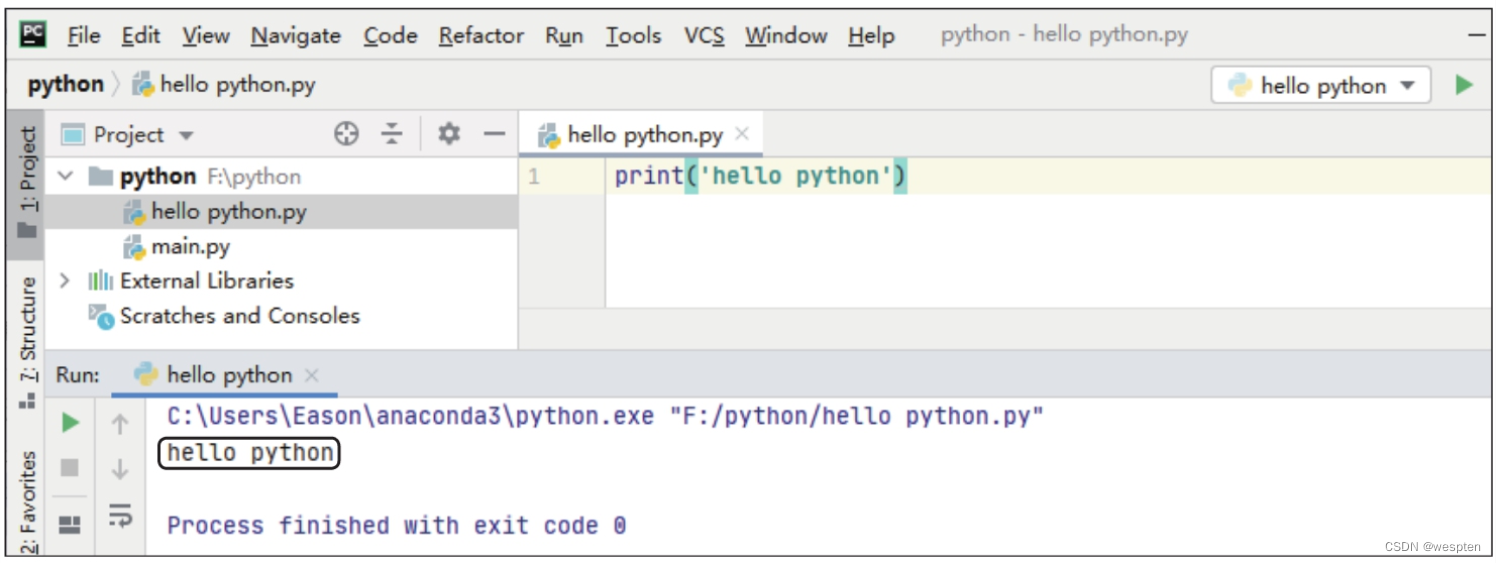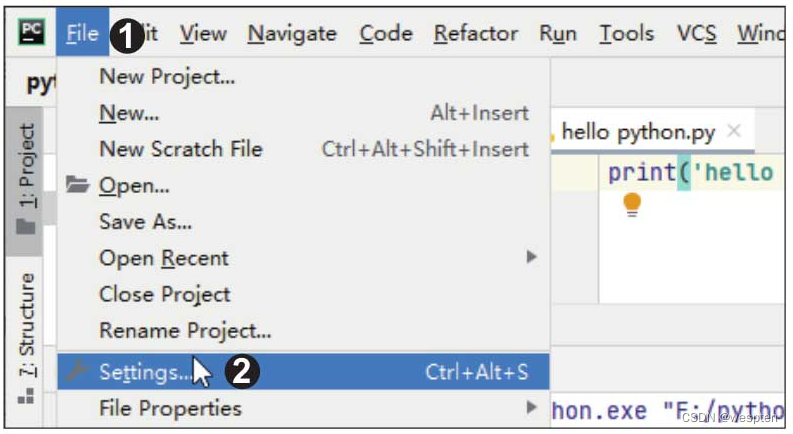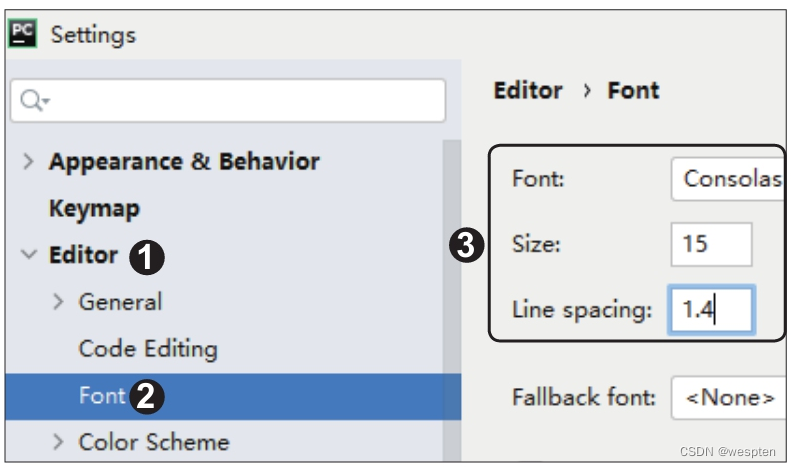### 2、Python的模块

Python最大的魅力之一就是拥有丰富的第三方模块，用户在编程时可以直接调用模块来实现强大的功能，无须自己编写复杂的代码。

1. 模块简介

1）内置模块

2）第三方的开源模块

Python之所以能风靡全球，其中一个很重要的原因就是它拥有很多第三方的开源模块，当我们要实现某种功能时就无须绞尽脑汁地编写基础代码，而是可以直接调用这些开源模块。第三方模块在使用前一般需要用户自行安装，而有些第三方模块会在安装编辑器（如PyCharm）时自动安装好。

3）自定义模块

Python用户可以将自己编写的代码或函数封装成模块，以方便在编写其他程序时调用，这样的模块就是自定义模块。需要注意的是，自定义模块不能和内置模块重名，否则将不能再导入内置模块。

2. 模块的安装

1）用pip命令安装模块

pip是Python提供的一个命令，主要功能就是安装和卸载第三方模块。用pip命令安装模块的方法最简单也最常用，这种方法默认将模块安装在Python安装目录中的“site-packages”文件夹下。下面来学习用pip命令安装模块的具体方法。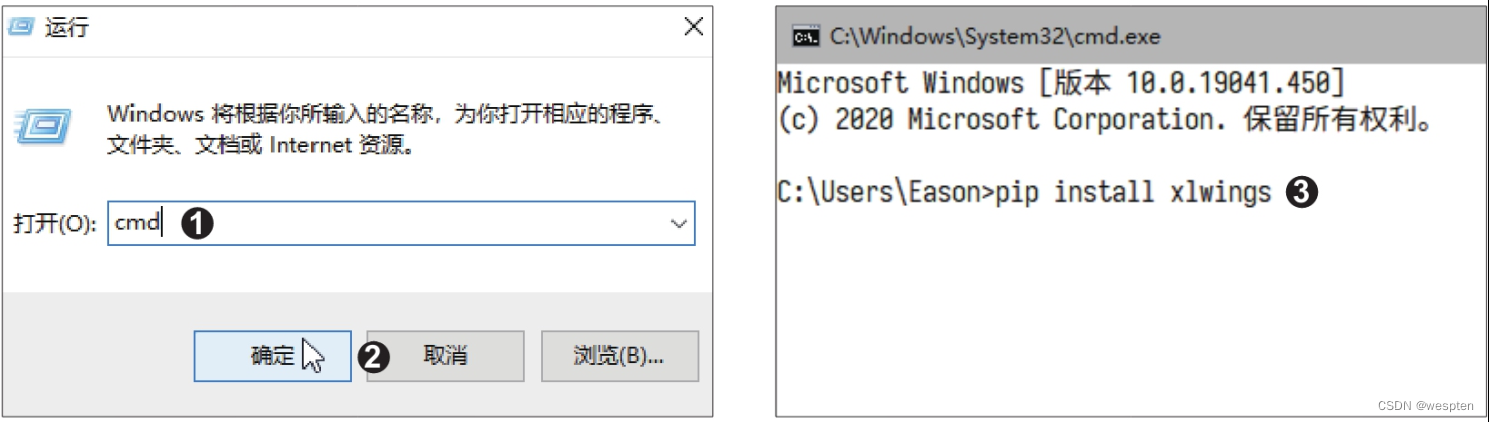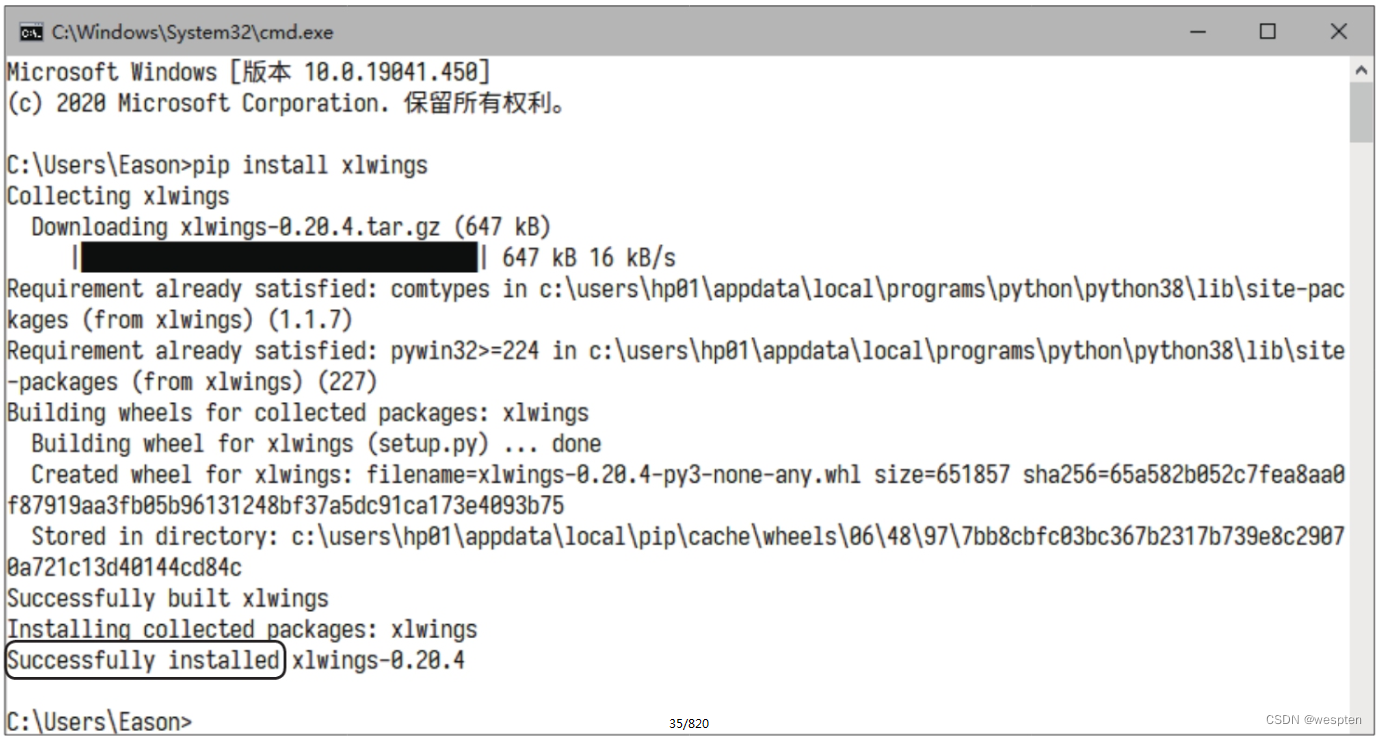pip命令下载模块时默认访问的服务器设在国外，速度不稳定，可能会导致安装失败，大家也可以通过国内的一些企业、院校、科研机构设立的镜像服务器来安装模块。

``pip install xlwings -i https://pypi.tuna.tsinghua.edu.cn/simple``

2）在PyCharm中安装模块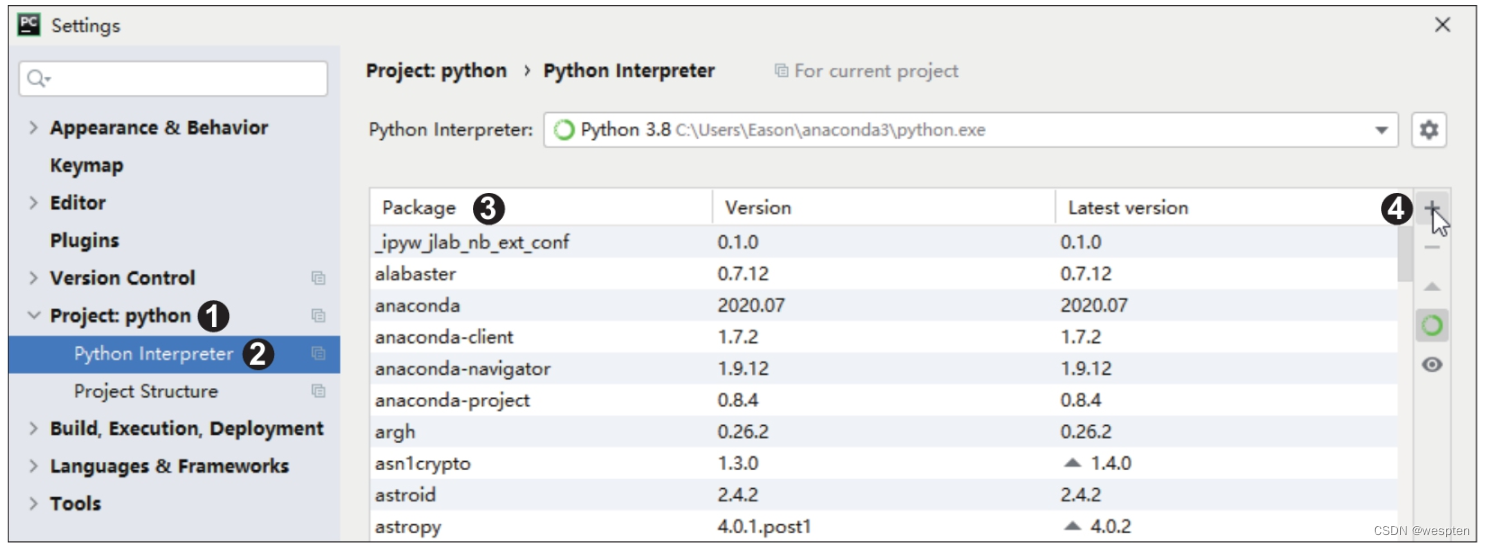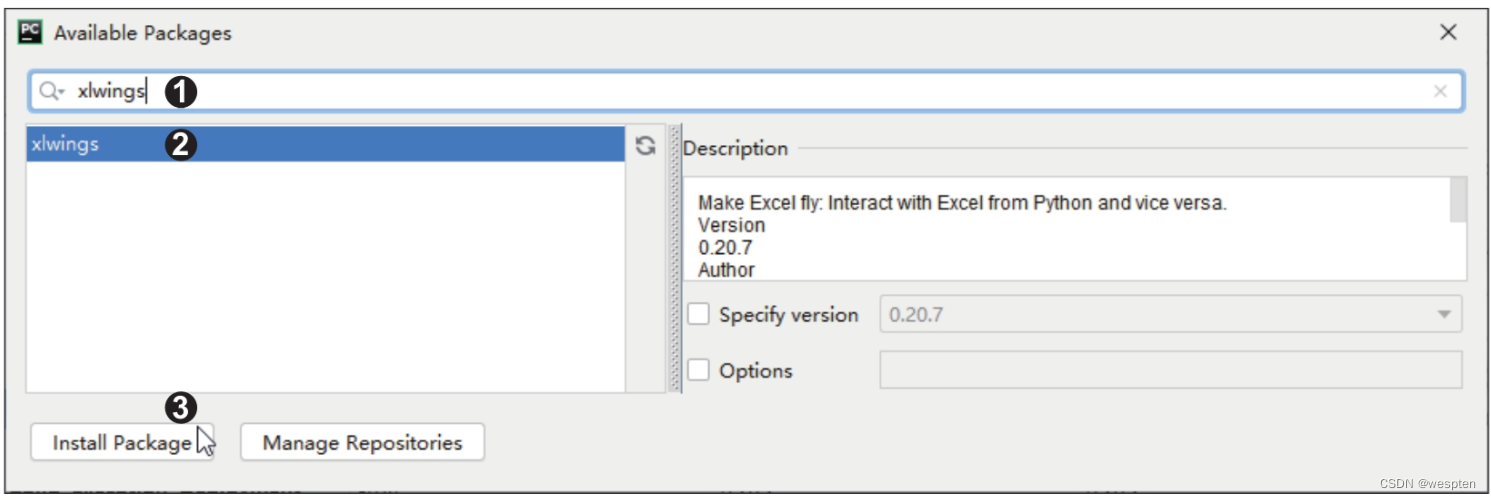3. 模块的导入

1）import语句导入法

import语句导入法是导入模块的常规方法。该方法会导入指定模块中的所有函数，适用于需要使用指定模块中的大量函数的情况。

import语句的基本语法格式如下：import 模块名

``import math  # 导入math模块import turtle  # 导入turtle模块``

``import matha = math.sqrt(16)print(a)``

``4.0``

2）from语句导入法

from语句的基本语法格式如下：from 模块名 import 函数名

``from math import sqrt  # 导入math模块中的单个函数from turtle import forward, backward, right, left  # 导入turtle模块中的多个函数``

``from math import sqrt　　# 导入math模块中的sqrt()函数a = sqrt(16)print(a)``

``4.0``

``import numpy as np  # 导入NumPy模块，并将其简写为npfrom math import factorial as fc  # 导入math模块中的factorial()函数，并将其简写为fc``

``from math import *  # 导入math模块中的所有函数a = sqrt(16)print(a)``

### 3、Python编码规范

1. 变量

● 变量名可以由任意数量的字母、数字、下划线组合而成，但是必须以字母或下划线开头，不能以数字开头。建议用英文字母开头，如a、b、c、a_1、b_1等。

● 不要用Python的保留字或内置函数来命名变量。例如，不要用import来命名变量，因为它是Python的保留字，有特殊的含义。

● 变量名对英文字母区分大小写。例如，D和d是两个不同的变量。

● 建议使用英文字母和数字组成变量名，并且变量名要有一定的意义，能直观地描述变量所代表的数据内容。例如，用变量name代表姓名数据，用变量age代表年龄数据，等等。

``x = 1print(x)y = x + 25print(y)``

``126``

2. 缩进

``1   x = 102   if x > 0:3       print('正数')4   else:5       print('负数')``

``1   让x等于102   如果x大于0：3       输出字符串'正数'4   否则：5       输出字符串'负数'``

3. 注释

1）单行注释

``1   a = 12   b = 23   if a == b:　　# 注意表达式里是两个等号4       print('a和b相等')5   else:6       print('a和b不相等')``

``1   a和b不相等``

``1   a = 12   b = 23   # 注意表达式里是两个等号4   if a == b:5       print('a和b相等')6   else:7       print('a和b不相等')``

• 单行注释放在被注释代码上方时，在“#”号之后先输入一个空格，再输入注释内容；
• 单行注释放在被注释代码后面时，“#”号和代码之间至少要有两个空格，“#”号与注释内容之间也要有一个空格。

2）多行注释

``1   '''2   这是多行注释，用3个单引号3   这是多行注释，用3个单引号4   这是多行注释，用3个单引号5   '''6   print('Hello, Python!')``

``1   Hello, Python!``

``1   """2   这是多行注释，用3个双引号3   这是多行注释，用3个双引号4   这是多行注释，用3个双引号5   """6   print('Hello, Python!')``

``1   Hello, Python!``

## 二、NumPy模块

NumPy模块是Python语言的一个科学计算的第三方模块，其名字由“Numerical Python”缩写而来。NumPy模块可以构建多维数据的容器，将各种类型的数据快速地整合在一起，完成多维数据的计算及大型矩阵的存储和处理。因此，Python中的很多模块都是在NumPy模块的基础上编写的。

### 1、创建数组

NumPy模块最主要的特点就是引入了数组的概念。数组是一些相同类型的数据的集合，这些数据按照一定的顺序排列，并且每个数据占用大小相同的存储空间。要使用数组组织数据，首先就要创建数组。NumPy模块提供多种创建数组的方法，创建的数组类型也多种多样，下面就来学习创建数组的方法。

1）使用array()函数创建数组

``1   import numpy as np2   a = np.array([1, 2, 3, 4])3   b = np.array(['产品编号', '销售数量', '销售单价', '销售金额'])4   print(a)5   print(b)``

``1   [1 2 3 4]2   ['产品编号' '销售数量' '销售单价' '销售金额']``

``1   import numpy as np2   c = np.array([[1, 2, 3], [4, 5, 6], [7, 8, 9]])3   print(c)``

``1   [[1 2 3]2    [4 5 6]3    [7 8 9]]``

``array(object, dtype=None, copy=True, order=None, subok=False, ndmin=0)``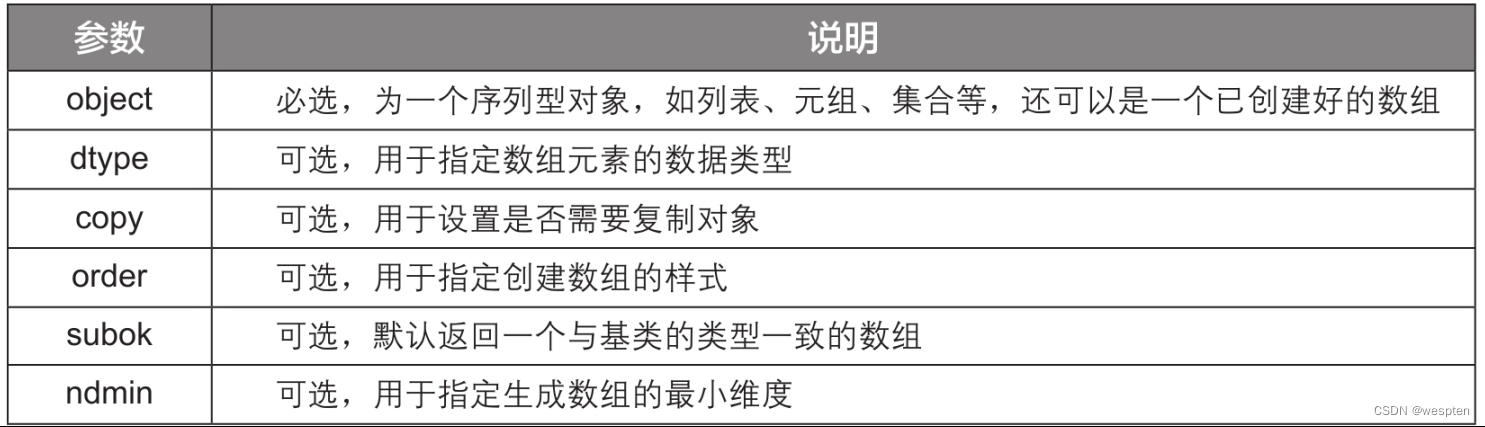2）创建等差数组

``1   import numpy as np2   d = np.arange(1, 20, 4)3   print(d)``

``1   [ 1 5 9 13 17]``

``1   import numpy as np2   d = np.arange(1, 20)3   print(d)``

``1   [ 1 2 3 4 5 6 7 8 9 10 11 12 13 14 15 16 17 18 19]``

``1   import numpy as np2   d = np.arange(20)3   print(d)``

``1   [ 0 1 2 3 4 5 6 7 8 9 10 11 12 13 14 15 16 17 18 19]``

``arange(start, stop, step, dtype=None)``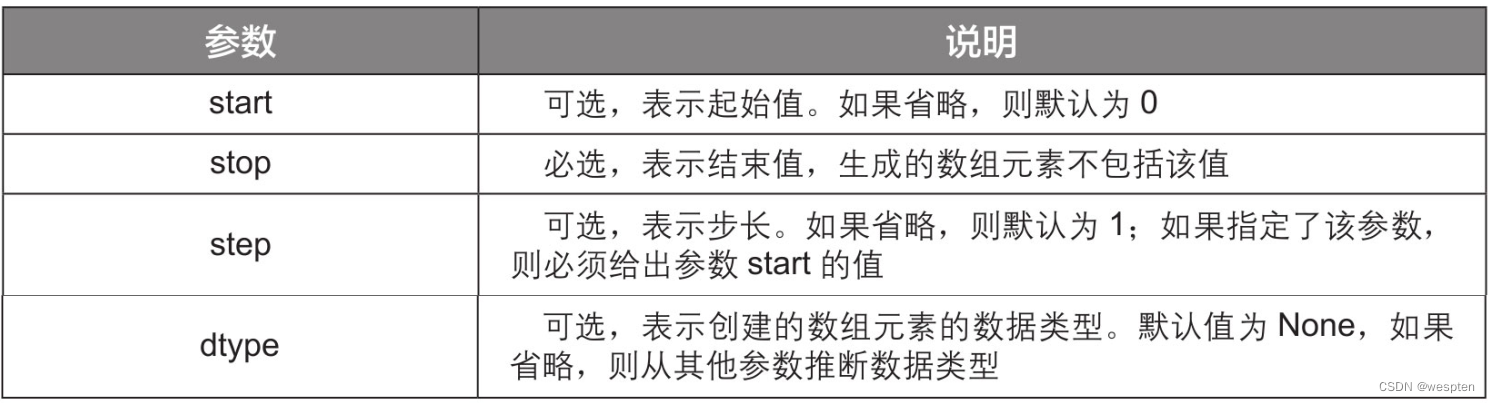3）创建随机数组

• rand()函数

``1   import numpy as np2   e = np.random.rand(3)3   print(e)``

``1   [0.52629764 0.60120953 0.928765  ]``

``1   import numpy as np2   e = np.random.rand(2, 3)3   print(e)``

``1   [[0.47760649 0.77905762 0.44320408]2    [0.33504138 0.95236697 0.12665502]]``
• randn()函数

``1   import numpy as np2   e = np.random.randn(3)3   print(e)``

``1   [0.53532424 1.21974306 0.16032314]``

``1   import numpy as np2   e = np.random.randn(3, 3)3   print(e)``

``1   [[ 0.28850844  0.9403601  -1.53854975]2    [-2.28107582  1.06579641  0.69591266]3    [-0.8554639  -0.28063843 -0.5227256 ]]``
• randint()函数

``1   import numpy as np2   e = np.random.randint(1, 5, 10)3   print(e)``

``1   [4 2 2 2 2 2 1 3 2 1]``

``1   import numpy as np2   e = np.random.randint(1, 10, (4, 2))3   print(e)``

``1   [[6 6]2    [9 4]3    [3 2]4    [9 7]]``

### 2、查看数组的属性

1）查看数组的行数和列数

``1   import numpy as np2   arr = np.array([[1, 2], [3, 4], [5, 6]])3   print(arr.shape)``

``1   (3, 2)``

``1   import numpy as np2   arr = np.array([[1, 2], [3, 4], [5, 6]])3   print(arr.shape)4   print(arr.shape)``

``1   32   2``

2）查看数组的元素个数

``1   import numpy as np2   arr = np.array([[1, 2], [3, 4], [5, 6]])3   print(arr.size)``

``1   6``

3）查看和转换数组元素的数据类型

``1   import numpy as np2   arr = np.array([[1.3, 2, 3.6, 4], [5, 6, 7.8, 8]])3   print(arr.dtype)``

``1   float64``

``1   import numpy as np2   arr = np.array([[1.3, 2, 3.6, 4], [5, 6, 7.8, 8]])3   arr1 = arr.astype(int)4   print(arr1)5   print(arr1.dtype)``

``1   [[1 2 3 4]2    [5 6 7 8]]3   int32``

4）查看数组的维数

``1   import numpy as np2   arr = np.array([[1, 2], [3, 4], [5, 6]])3   print(arr.ndim)``

``1   2``

### 3、选取数组元素

1. 一维数组的元素选取

1）选取单个元素

``1   import numpy as np2   arr = np.array([12, 2, 40, 64, 56, 6, 57, 18, 95, 17, 21, 12])3   print(arr)4   print(arr)5   print(arr[-1])6   print(arr[-4])``

``1   122   63   124   95``

2）选取连续的元素

``1   import numpy as np2   arr = np.array([12, 2, 40, 64, 56, 6, 57, 18, 95, 17, 21, 12])3   print(arr[1:6])4   print(arr[3:-2])5   print(arr[:3])6   print(arr[:-3])7   print(arr[3:])8   print(arr[-3:])``

``1   [ 2 40 64 56 6]2   [64 56 6 57 18 95 17]3   [12 2 40]4   [12 2 40 64 56 6 57 18 95]5   [64 56 6 57 18 95 17 21 12]6   [17 21 12]``

3）选取不连续的元素

``1   import numpy as np2   arr = np.array([12, 2, 40, 64, 56, 6, 57, 18, 95, 17, 21, 12])3   print(arr[1:5:2])4   print(arr[5:1:-2])5   print(arr[::3])6   print(arr[3::])7   print(arr[:3:])``

``1   [ 2 64]2   [ 6 64]3   [12 64 57 17]4   [64 56 6 57 18 95 17 21 12]5   [12 2 40]``

2. 二维数组的元素选取

1）选取单个元素

``1   import numpy as np2   arr = np.array([[1, 2, 3], [4, 5, 6], [7, 8, 9], [10, 11, 12]])3   print(arr[1, 2])``

``1   6``

2）选取单行或单列的元素

``1   import numpy as np2   arr = np.array([[1, 2, 3], [4, 5, 6], [7, 8, 9], [10, 11, 12]])3   print(arr)4   print(arr[:, 1])``

``1   [7 8 9]2   [ 2 5 8 11]``

3）选取某些行或某些列的元素

``1   import numpy as np2   arr = np.array([[1, 2, 3], [4, 5, 6], [7, 8, 9], [10, 11, 12]])3   print(arr[1:3])4   print(arr[:2])5   print(arr[2:])``

``1   [[4 5 6]2    [7 8 9]]3   [[1 2 3]4    [4 5 6]]5   [[7 8 9]6    [10 11 12]]``

``1   import numpy as np2   arr = np.array([[1, 2, 3, 4], [5, 6, 7, 8], [9, 10, 11, 12], [13, 14, 15, 16]])3   print(arr[:, 1:3])4   print(arr[:, :2])5   print(arr[:, 2:])``

``1   [[ 2 3]2    [ 6 7]3    [10 11]4    [14 15]]5   [[ 1 2]6    [ 5 6]7    [ 9 10]8    [13 14]]9   [[3 4]10    [7 8]11    [11 12]12    [15 16]]``

4）同时选取行列元素

``1   import numpy as np2   arr = np.array([[1, 2, 3, 4], [5, 6, 7, 8], [9, 10, 11, 12], [13, 14, 15, 16]])3   print(arr[0:2, 1:3])``

``1   [[2 3]2    [6 7]]``

### 4、数组的重塑与转置

1. 一维数组的重塑

NumPy模块中的reshape()函数可以在不改变数组元素内容和个数的情况下重塑数组的形状。下面先从较简单的一维数组的重塑开始讲解。一维数组的重塑就是将一行或一列的数组转换为多行多列的数组，演示代码如下：

``1   import numpy as np2   arr = np.array([1, 2, 3, 4, 5, 6, 7, 8])3   a = arr.reshape(2, 4)4   b = arr.reshape(4, 2)5   print(a)6   print(b)``

``1   [[1 2 3 4]2    [5 6 7 8]]3   [[1 2]4    [3 4]5    [5 6]6    [7 8]]``

2. 多维数组的重塑

reshape()函数除了可以将一维数组转换为多维数组，还可以更改多维数组的形状。演示代码如下：

``1   import numpy as np2   arr = np.array([[1, 2, 3, 4], [5, 6, 7, 8], [9, 10, 11, 12]])3   c = arr.reshape(4, 3)4   d = arr.reshape(2, 6)5   print(c)6   print(d)``

``1   [[ 1 2 3]2    [ 4 5 6]3    [ 7 8 9]4    [10 11 12]]5   [[ 1 2 3 4 5 6]6    [ 7 8 9 10 11 12]]``

``1   import numpy as np2   arr = np.array([[1, 2, 3, 4], [5, 6, 7, 8], [9, 10, 11, 12]])3   print(arr.flatten())4   print(arr.ravel())``

``1   [ 1  2  3  4  5  6  7  8  9 10 11 12]2   [ 1  2  3  4  5  6  7  8  9 10 11 12]``

3. 数组的转置

1）T属性

T属性的用法很简单，只需在要转置的数组后调用T属性即可。

``1   import numpy as np2   arr = np.array([[1, 2, 3, 4], [5, 6, 7, 8], [9, 10, 11, 12]])3   print(arr)4   print(arr.T)``

``1   [[ 1 2 3 4]2    [ 5 6 7 8]3    [ 9 10 11 12]]4   [[ 1 5 9]5    [ 2 6 10]6    [ 3 7 11]7    [ 4 8 12]]``

2）transpose()函数

transpose()函数是通过调换数组的行和列的索引值来转置数组的。

``1   import numpy as np2   arr = np.array([[1, 2, 3, 4], [5, 6, 7, 8], [9, 10, 11, 12]])3   arr1 = np.transpose(arr)4   print(arr1)``

``1   [[ 1 5  9]2    [ 2 6 10]3    [ 3 7 11]4    [ 4 8 12]]``

### 5、数组的处理

1. 添加数组元素

1）append()函数

append()函数可以在数组的末尾添加元素。

``1   import numpy as np2   arr = np.array([[1, 2, 3], [4, 5, 6]])3   arr1 = np.append(arr, [[7, 8, 9]])4   print(arr1)``

``1   [1 2 3 4 5 6 7 8 9]``

``1   import numpy as np2   arr = np.array([[1, 2, 3], [4, 5, 6]])3   arr1 = np.append(arr, [[7, 8, 9]], axis=0)4   print(arr1)``

``1   [[1 2 3]2    [4 5 6]3    [7 8 9]]``

append()函数的参数axis也可以设置为1，表示元素会添加在数组的列方向上，也就是说，数组的列数会增加，而行数不变。

``1   import numpy as np2   arr = np.array([[1, 2, 3], [4, 5, 6]])3   arr1 = np.append(arr, [[7, 8], [9, 10]], axis=1)4   print(arr1)``

``1   [[ 1  2  3  7  8]2    [ 4  5  6  9  10]]``

``append(arr, values, axis=None)``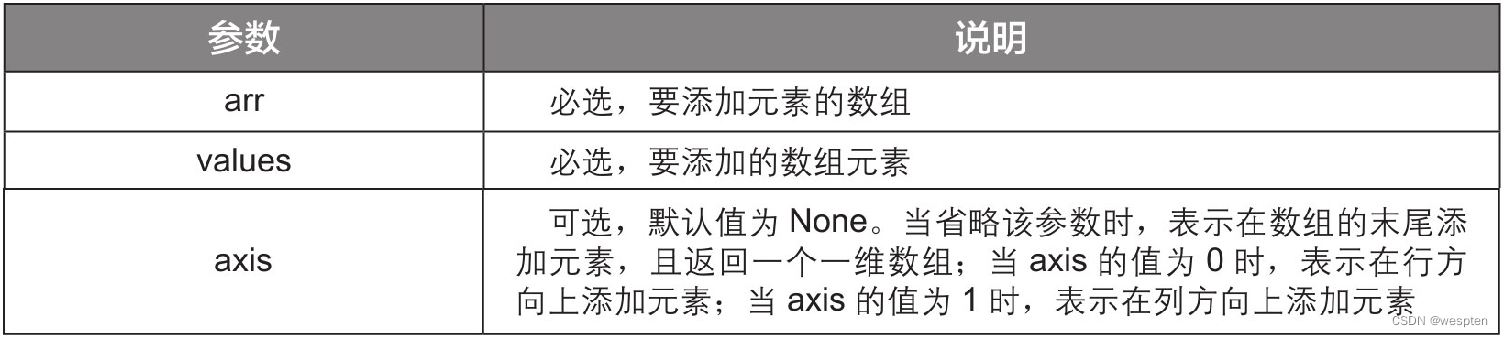2）insert()函数

insert()函数用于在数组的指定位置插入元素。

``1   import numpy as np2   arr = np.array([[1, 2], [3, 4], [5, 6]])3   arr1 = np.insert(arr, 1, [7, 8])4   print(arr1)``

``1   [1 7 8 2 3 4 5 6]``

``1   import numpy as np2   arr = np.array([[1, 2], [3, 4], [5, 6]])3   arr1 = np.insert(arr, 1, [7, 8], axis=0)4   arr2 = np.insert(arr, 1, [7, 8, 9], axis=1)5   print(arr1)6   print(arr2)``

``1   [[1 2]2    [7 8]3    [3 4]4    [5 6]]5   [[1 7 2]6    [3 8 4]7    [5 9 6]]``

``insert(arr, obj, values, axis)``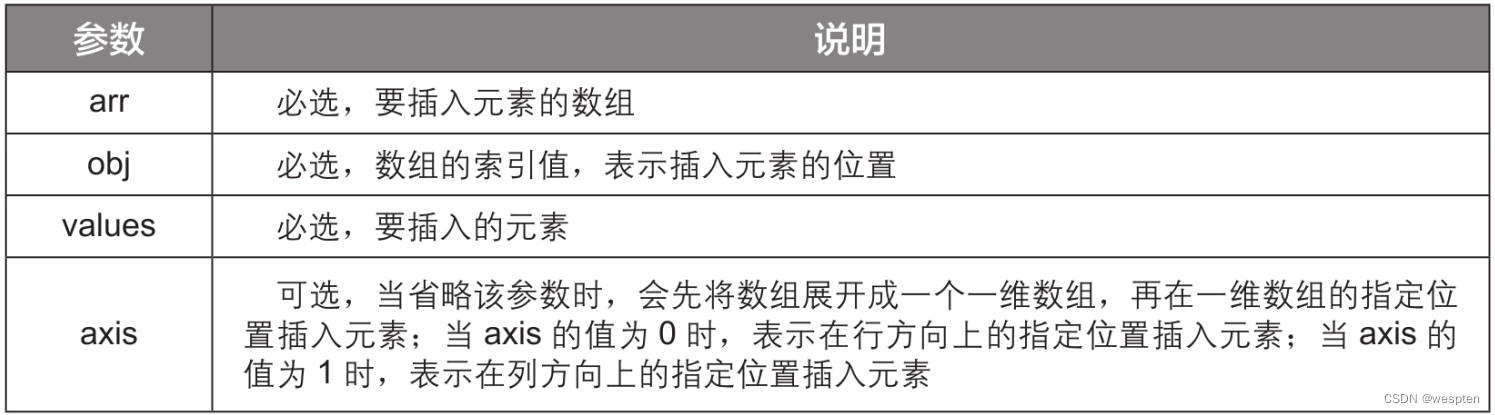2. 删除数组元素

``1   import numpy as np2   arr = np.array([[1, 2, 3], [4, 5, 6], [7, 8, 9]])3   arr1 = np.delete(arr, 2)4   arr2 = np.delete(arr, 2, axis=0)5   arr3 = np.delete(arr, 2, axis=1)6   print(arr1)7   print(arr2)8   print(arr3)``

``1   [1 2 4 5 6 7 8 9]2   [[1 2 3]3    [4 5 6]]4   [[1 2]5    [4 5]6    [7 8]]``

3. 处理数组的缺失值

``1   import numpy as np2   arr = np.array([1, 2, 3, 4, 5, 6, np.nan, 8, 9])3   print(arr)4   print(np.isnan(arr))``

``1   [ 1.  2.  3.  4.  5.  6. nan  8.  9.]2   [False False False False False False  True False False]``

``1   arr[np.isnan(arr)] = 02   print(arr)``

``1   [1. 2. 3. 4. 5. 6. 0. 8. 9.]``

4. 处理数组的重复值

``1   import numpy as np2   arr = np.array([8, 4, 2, 3, 5, 2, 5, 5, 6, 8, 8, 9])3   arr1 = np.unique(arr)4   arr1, arr2 = np.unique(arr, return_counts=True)5   print(arr1)6   print(arr2)``

``1   [2 3 4 5 6 8 9]2   [2 1 1 3 1 3 1]``

5. 拼接数组

1）concatenate()函数

concatenate()函数能一次合并多个数组，是数组拼接最常用的方法。

``1   import numpy as np2   arr1 = np.array([[1, 2, 3], [4, 5, 6]])3   arr2 = np.array([[7, 8, 9], [10, 11, 12]])4   arr3 = np.concatenate((arr1, arr2), axis=0)5   arr4 = np.concatenate((arr1, arr2), axis=1)6   print(arr3)7   print(arr4)``

``1   [[ 1  2  3]2    [ 4  5  6]3    [ 7  8  9]4    [10 11 12]]5   [[ 1  2  3  7  8  9]6    [ 4  5  6 10 11 12]]``

2）hstack()函数和vstack()函数

hstack()函数能以水平堆叠的方式拼接数组。

``1   import numpy as np2   arr1 = np.array([[1, 2, 3], [4, 5, 6]])3   arr2 = np.array([[7, 8, 9], [10, 11, 12]])4   arr3 = np.hstack((arr1, arr2))5   print(arr3)``

``1   [[ 1  2  3  7  8  9]2    [ 4  5  6 10 11 12]]``

vstack()函数能以垂直堆叠的方式拼接数组。

``1   import numpy as np2   arr1 = np.array([[1, 2, 3], [4, 5, 6]])3   arr2 = np.array([[7, 8, 9], [10, 11, 12]])4   arr3 = np.vstack((arr1, arr2))5   print(arr3)``

``1   [[ 1  2  3]2    [ 4  5  6]3    [ 7  8  9]4    [10 11 12]]``

6. 拆分数组

1）split()函数

split()函数可以按指定的份数将一个数组均分为多个数组。

``1   import numpy as np2   arr = np.array([1, 2, 3, 4, 5, 6, 7, 8, 9, 10, 11, 12])3   arr1 = np.split(arr, 2)4   arr2 = np.split(arr, 4)5   print(arr1)6   print(arr2)``

``1   [array([1, 2, 3, 4, 5, 6]), array([ 7,  8,  9, 10, 11, 12])]2   [array([1, 2, 3]), array([4, 5, 6]), array([7, 8, 9]), array([10, 11, 12])]``

split()函数除了可以均分数组，还可以按照指定的索引位置拆分数组，此时需要将split()函数的第2个参数设置为一个数组。

``1   import numpy as np2   arr = np.array([1, 2, 3, 4, 5, 6, 7, 8, 9, 10, 11, 12])3   arr3 = np.split(arr, [2, 6])4   arr4 = np.split(arr, [2, 3, 8, 10])5   print(arr3)6   print(arr4)``

``1   [array([1, 2]), array([3, 4, 5, 6]), array([ 7, 8, 9, 10, 11, 12])]2   [array([1, 2]), array(), array([4, 5, 6, 7, 8]), array([ 9, 10]), array([11, 12])]``

2）hsplit()函数和vsplit()函数

hsplit()函数能将一个数组横向拆分为多个数组。vsplit()函数能将一个数组纵向拆分为多个数组。演示代码如下：

``1   import numpy as np2   arr = np.array([[1, 2, 3, 4], [5, 6, 7, 8], [9, 10, 11, 12], [13, 14, 15, 16]])3   arr5 = np.hsplit(arr, 2)4   arr6 = np.vsplit(arr, 2)5   print(arr5)6   print(arr6)``

``1   [array([[ 1,  2],2          [ 5,  6],3          [ 9, 10],4          [13, 14]]), array([[ 3,  4],5          [ 7,  8],6          [11, 12],7          [15, 16]])]8   [array([[1, 2, 3, 4],9          [5, 6, 7, 8]]), array([[ 9, 10, 11, 12],10          [13, 14, 15, 16]])]``

### 6、数组的运算

NumPy模块的优势不仅在于支持多个维度数据的存储和展示，它还能很好地支持数组的数学运算，如数组之间的四则运算和数组元素的统计运算。

1. 数组之间的四则运算

``1   import numpy as np2   arr1 = np.array([[1, 2, 3, 4], [5, 6, 7, 8]])3   arr2 = np.array([[9, 10, 11, 12], [13, 14, 15, 16]])4   arr3 = arr1 + arr25   arr4 = arr1 * arr26   print(arr3)7   print(arr4)``

``1   [[10 12 14 16]2    [18 20 22 24]]3   [[ 9 20 33 48]4    [ 65  84 105 128]]``

``1   import numpy as np2   arr = np.array([[1, 2, 3, 4], [5, 6, 7, 8]])3   arr5 = arr + 54   arr6 = arr * 105   print(arr5)6   print(arr6)``

``1   [[ 6 7 8 9]2    [10 11 12 13]]3   [[10 20 30 40]4    [50 60 70 80]]``

2. 数组元素的统计运算

1）求和

sum()函数用于求和，它除了能对整个数组的所有元素求和，还能对数组的每一行或每一列元素分别求和。

``1   import numpy as np2   arr = np.array([[1, 2, 3, 4], [5, 6, 7, 8], [9, 10, 11, 12]])3   arr1 = arr.sum()4   arr2 = arr.sum(axis=0)5   arr3 = arr.sum(axis=1)6   print(arr1)7   print(arr2)8   print(arr3)``

``1   782   [15 18 21 24]3   [10 26 42]``

2）求平均值

mean()函数用于求平均值，它除了能对整个数组的所有元素求平均值，还能对数组的每一行或每一列元素分别求平均值。

``1   import numpy as np2   arr = np.array([[1, 2, 3, 4], [5, 6, 7, 8], [9, 10, 11, 12]])3   arr1 = arr.mean()4   arr2 = arr.mean(axis=0)5   arr3 = arr.mean(axis=1)6   print(arr1)7   print(arr2)8   print(arr3)``

``1   6.52   [5. 6. 7. 8.]3   [ 2.5  6.5 10.5]``

3）求最值

max()函数和min()函数分别用于求数组元素的最大值和最小值。与sum()函数和mean()函数类似，max()函数和min()函数也可以通过设置参数来指定求最值的方式。下面以max()函数为例进行讲解，演示代码如下：

``1   import numpy as np2   arr = np.array([[1, 2, 3, 4], [5, 6, 7, 8], [9, 10, 11, 12]])3   arr1 = arr.max()4   arr2 = arr.max(axis=0)5   arr3 = arr.max(axis=1)6   print(arr1)7   print(arr2)8   print(arr3)``

``1   122   [ 9 10 11 12]3   [ 4 8 12]``

## 三、pandas模块

pandas模块是基于NumPy模块开发的，它不仅能直观地展现数据的结构，还具备强大的数据处理和分析功能。从某种程度上来说，pandas模块是Python成为强大而高效的数据分析工具的重要因素之一。

### 1、数据结构

pandas模块中有两个重要的数据结构对象——Series和DataFrame。使用这两个数据结构对象可以在计算机的内存中构建虚拟的数据库。下面一起来学习如何使用这两个对象创建数据结构。

1. Series对象

Series是一种类似于NumPy模块创建的一维数组的对象，与一维数组不同的是，Series对象不仅包含数据元素，还包含一组与数据元素对应的行标签。

``1   import pandas as pd2   s = pd.Series(['短裤', '毛衣', '连衣裙', '牛仔裤'])3   print(s)``

``1   0     短裤2   1     毛衣3   2    连衣裙4   3    牛仔裤5   dtype: object``

``1   import pandas as pd2   s1 = pd.Series(['短裤', '毛衣', '连衣裙', '牛仔裤'], index=['a001', 'a002', 'a003', 'a004'])3   print(s1)``

``1   a001     短裤2   a002     毛衣3   a003    连衣裙4   a004    牛仔裤5   dtype: object``

``1   import pandas as pd2   s2 = pd.Series({'a001': '短裤', 'a002': '毛衣', 'a003': '连衣裙', 'a004': '牛仔裤'})3   print(s2)``

``1   a001     短裤2   a002     毛衣3   a003    连衣裙4   a004    牛仔裤5   dtype: object``

2. DataFrame对象

DataFrame是一种二维的数据结构对象，用该对象创建的数据结构在形式上类似于Excel表格。相比Series对象，DataFrame对象在实际工作中的应用更为广泛，因此，后续章节与pandas模块相关的内容都主要围绕DataFrame对象展开。先来学习使用DataFrame对象基于列表创建数据结构的方法，演示代码如下：

``1   import pandas as pd2   df = pd.DataFrame([['短裤', 45], ['毛衣', 69], ['连衣裙', 119], ['牛仔裤', 99]])3   print(df)``

``1           0     12   0     短裤    453   1     毛衣    694   2   连衣裙   1195   3   牛仔裤    99``

``1   import pandas as pd2   df1 = pd.DataFrame([['短裤', 45], ['毛衣', 69], ['连衣裙', 119], ['牛仔裤', 99]], columns=['产品', '单价'], index=['a001', 'a002', 'a003', 'a004'])3   print(df1)``

``1            产品  单价2   a001     短裤    453   a002     毛衣    694   a003   连衣裙   1195   a004   牛仔裤    99``

``1   import pandas as pd2   df2 = pd.DataFrame({'产品': ['短裤', '毛衣', '连衣裙', '牛仔裤'], '单价': [45, 69, 119, 99]})3   print(df2)``

``1         产品   单价2   0     短裤     453   1     毛衣     694   2   连衣裙    1195   3   牛仔裤     99``

``1   import pandas as pd2   df3 = pd.DataFrame({'产品': ['短裤', '毛衣', '连衣裙', '牛仔裤'], '单价': [45, 69, 119, 99]}, index=['a001', 'a002', 'a003', 'a004'])3   print(df3)``

``1            产品  单价2   a001     短裤    453   a002     毛衣    694   a003   连衣裙   1195   a004   牛仔裤    99``

### 2、读取数据

1. 读取Excel工作簿数据

1）读取某个工作表的数据

``1   import pandas as pd2   data = pd.read_excel('订单表.xlsx', sheet_name=3)3   print(data)``

``1      订单编号     产品  数量   金额2   0     d001   投影仪   5台   20003   1     d002   马克笔   5盒    3004   2     d003   打印机   1台    2985   3     d004   点钞机   1台    3496   4     d005   复印纸   2箱    1007   5     d006   条码纸   6卷     34``

2）指定读取数据的列标签

``1   import pandas as pd2   data = pd.read_excel('订单表.xlsx', sheet_name=3, header=0)3   print(data) ``

``1     订单编号     产品  数量   金额2   0     d001   投影仪   5台   20003   1     d002   马克笔   5盒    3004   2     d003   打印机   1台    2985   3     d004   点钞机   1台    3496   4     d005   复印纸   2箱    1007   5     d006   条码纸   6卷     34``

``1   import pandas as pd2   data = pd.read_excel('订单表.xlsx', sheet_name=3, header=1)3   print(data)``

``1       d001   投影仪   5台   20002   0   d002   马克笔   5盒    3003   1   d003   打印机   1台    2984   2   d004   点钞机   1台    3495   3   d005   复印纸   2箱    1006   4   d006   条码纸   6卷     34``

``1   import pandas as pd2   data = pd.read_excel('订单表.xlsx', sheet_name=3, header=None)3   print(data)``

``1             0       1     2      32   0   订单编号     产品  数量   金额3   1      d001   投影仪   5台   20004   2      d002   马克笔   5盒    3005   3      d003   打印机   1台    2986   4      d004   点钞机   1台    3497   5      d005   复印纸   2箱    1008   6      d006   条码纸   6卷     34``

3）指定读取数据的行标签

``1   import pandas as pd2   data = pd.read_excel('订单表.xlsx', sheet_name=3, index_col=0)3   print(data)``

``1               产品  数量   金额2   订单编号3      d001   投影仪   5台   20004      d002   马克笔   5盒    3005      d003   打印机   1台    2986      d004   点钞机   1台    3497      d005   复印纸   2箱    1008      d006   条码纸   6卷     34``

``1   import pandas as pd2   data = pd.read_excel('订单表.xlsx', sheet_name=3, index_col=1)3   print(data)``

``1          订单编号  数量   金额2     产品3   投影仪     d001   5台   20004   马克笔     d002   5盒    3005   打印机     d003   1台    2986   点钞机     d004   1台    3497   复印纸     d005   2箱    1008   条码纸     d006   6卷     34``

4）读取指定列

``1   import pandas as pd2   data = pd.read_excel('订单表.xlsx', sheet_name=3, usecols=)3   print(data)``

``1       数量2   0    5台3   1    5盒4   2    1台5   3    1台6   4    2箱7   5    6卷``

``1   import pandas as pd2   data = pd.read_excel('订单表.xlsx', sheet_name=3, usecols=[1, 3])3   print(data)``

``1         产品    金额2   0   投影仪   20003   1   马克笔    3004   2   打印机    2985   3   点钞机    3496   4   复印纸    1007   5   条码纸     34``

2. 读取csv文件数据

csv是一种存储数据的文件格式，其本质上是一个文本文件，只能存储文本，不能存储格式、公式、宏等，所以所占存储空间通常较小。csv文件一般用逗号分隔一系列值，它既可以用Excel程序打开，也可以用文本编辑器（如记事本）打开。

``1   import pandas as pd2   data = pd.read_csv('订单表.csv')3   print(data)``

``1      订单编号     产品   数量   金额2   0      d001   投影仪    5台   20003   1      d002   马克笔    5盒    3004   2      d003   打印机    1台    2985   3      d004   点钞机    1台    3496   4      d005   复印纸    2箱    1007   5      d006   条码纸    6卷     34``

``1   import pandas as pd2   data = pd.read_csv('订单表.csv', nrows=3)3   print(data)``

``1      订单编号     产品   数量   金额2   0      d001   投影仪    5台   20003   1      d002   马克笔    5盒    3004   2      d003   打印机    1台    298``

### 3、查看数据

1. 查看数据的前几行

``1   import pandas as pd2   data = pd.read_excel('订单表.xlsx', sheet_name=3)3   print(data.head())``

``1      订单编号     产品   数量   金额2   0      d001   投影仪    5台   20003   1      d002   马克笔    5盒    3004   2      d003   打印机    1台    2985   3      d004   点钞机    1台    3496   4      d005   复印纸    2箱    100``

``1   import pandas as pd2   data = pd.read_excel('订单表.xlsx', sheet_name=3)3   print(data.head(3))``

``1      订单编号     产品   数量   金额2   0      d001   投影仪    5台   20003   1      d002   马克笔    5盒    3004   2      d003   打印机    1台    298``

2. 查看数据的行数和列数

``1   import pandas as pd2   data = pd.read_excel('订单表.xlsx', sheet_name=3)3   print(data)4   print(data.shape)``

``1      订单编号     产品   数量   金额2   0      d001   投影仪    5台   20003   1      d002   马克笔    5盒    3004   2      d003   打印机    1台    2985   3      d004   点钞机    1台    3496   4      d005   复印纸    2箱    1007   5      d006   条码纸    6卷     348   (6, 4)``

3. 查看数据的类型

``1   import pandas as pd2   data = pd.read_excel('订单表.xlsx', sheet_name=3)3   print(data.info())``

``1   <class 'pandas.core.frame.DataFrame'>2   RangeIndex: 6 entries, 0 to 53   Data columns (total 4 columns):4   订单编号    6 non-null object5   产品      6 non-null object6   数量      6 non-null object7   金额      6 non-null int648   dtypes: int64(1), object(3)9   memory usage: 320.0+ bytes10   None``

``1   import pandas as pd2   data = pd.read_excel('订单表.xlsx', sheet_name=3)3   print(data['金额'].dtype)``

``1   int64``

``1   import pandas as pd2   data = pd.read_excel('订单表.xlsx', sheet_name=3)3   data['金额'] = data['金额'].astype('float64')4   print(data)5   print(data['金额'].dtype)``

``1      订单编号     产品   数量     金额2   0      d001   投影仪    5台   2000.03   1      d002   马克笔    5盒    300.04   2      d003   打印机    1台    298.05   3      d004   点钞机    1台    349.06   4      d005   复印纸    2箱    100.07   5      d006   条码纸    6卷     34.08   float64``

### 4、选择数据

1. 选择行数据

``1   import pandas as pd2   data = pd.read_excel('订单表.xlsx', sheet_name=3, index_col=0)3   print(data)``

``1               产品  数量   金额2   订单编号3      d001   投影仪   5台   20004      d002   马克笔   5盒    3005      d003   打印机   1台    2986      d004   点钞机   1台    3497      d005   复印纸   2箱    1008      d006   条码纸   6卷     34``

1）选择单行数据

``1   import pandas as pd2   data = pd.read_excel('订单表.xlsx', sheet_name=3, index_col=0)3   print(data.loc['d001'])``

``1   产品     投影仪2   数量     5台3   金额     20004   Name: d001, dtype: object``

``1   import pandas as pd2   data = pd.read_excel('订单表.xlsx', sheet_name=3, index_col=0)3   print(data.iloc)``

``1   产品    打印机2   数量    1台3   金额    2984   Name: d003, dtype: object``

2）选择多行数据

loc方法和iloc方法除了能选择单行数据外，还能选择多行数据。

``1   import pandas as pd2   data = pd.read_excel('订单表.xlsx', sheet_name=3, index_col=0)3   print(data.loc[['d002', 'd004']])``

``1               产品  数量   金额2   订单编号3      d002   马克笔   5盒   3004      d004   点钞机   1台   349``

``1   import pandas as pd2   data = pd.read_excel('订单表.xlsx', sheet_name=3, index_col=0)3   print(data.iloc[[1, 5]])``

``1               产品   数量   金额2   订单编号3      d002   马克笔    5盒  3004      d006   条码纸    6卷   34``

``1   import pandas as pd2   data = pd.read_excel('订单表.xlsx', sheet_name=3, index_col=0)3   print(data.iloc[1:5])``

``1               产品   数量   金额2   订单编号3      d002   马克笔    5盒   3004      d003   打印机    1台   2985      d004   点钞机    1台   3496      d005   复印纸    2箱   100``

3）选择满足条件的行

``1   import pandas as pd2   data = pd.read_excel('订单表.xlsx', sheet_name=3, index_col=0)3   a = data['金额'] < 3004   print(data[a])``

``1               产品   数量   金额2   订单编号3      d003   打印机    1台   2984      d005   复印纸    2箱   1005      d006   条码纸    6卷    34``

2. 选择列数据

``1   import pandas as pd2   data = pd.read_excel('订单表.xlsx', sheet_name=3)3   print(data)``

``1       订单编号    产品   数量   金额2   0      d001   投影仪    5台   20003   1      d002   马克笔    5盒    3004   2      d003   打印机    1台    2985   3      d004   点钞机    1台    3496   4      d005   复印纸    2箱    1007   5      d006   条码纸    6卷     34``

1）选择单列数据

``1   import pandas as pd2   data = pd.read_excel('订单表.xlsx', sheet_name=3)3   print(data['产品'])``

``1   0    投影仪2   1    马克笔3   2    打印机4   3    点钞机5   4    复印纸6   5    条码纸7   Name: 产品, dtype: object``

2）选择多列数据

``1   import pandas as pd2   data = pd.read_excel('订单表.xlsx', sheet_name=3)3   print(data[['产品', '金额']])``

``1         产品   金额2   0   投影仪   20003   1   马克笔    3004   2   打印机    2985   3   点钞机    3496   4   复印纸    1007   5   条码纸     34``

``1   import pandas as pd2   data = pd.read_excel('订单表.xlsx', sheet_name=3)3   print(data.iloc[:, [1, 3]])``

``1        产品    金额2   0   投影仪   20003   1   马克笔    3004   2   打印机    2985   3   点钞机    3496   4   复印纸    1007   5   条码纸     34``

``1   import pandas as pd2   data = pd.read_excel('订单表.xlsx', sheet_name=3)3   print(data.iloc[:, 1:3])``

``1        产品    数量2   0   投影仪    5台3   1   马克笔    5盒4   2   打印机    1台5   3   点钞机    1台6   4   复印纸    2箱7   5   条码纸    6卷 ``

3. 同时选择行列数据

``1   import pandas as pd2   data = pd.read_excel('订单表.xlsx', sheet_name=3, index_col=0)3   data1 = data.loc[['d001', 'd005'], ['产品', '金额']]4   print(data1)``

``1               产品   金额2   订单编号3      d001   投影仪   20004      d005   复印纸    100``

``1   import pandas as pd2   data = pd.read_excel('订单表.xlsx', sheet_name=3, index_col=0)3   data2 = data.iloc[[2, 4], [0, 2]]4   print(data2)``

``1               产品   金额2   订单编号3      d003   打印机    2984      d005   复印纸    100``

### 5、修改行标签和列标签

``1       订单编号    产品   数量   金额2   0      d001   投影仪    5台   20003   1      d002   马克笔    5盒    3004   2      d003   打印机    1台    2985   3      d004   点钞机    1台    3496   4      d005   复印纸    2箱    1007   5      d006   条码纸    6卷     34``

``1   import pandas as pd2   data = pd.read_excel('订单表.xlsx', sheet_name=3)3   print(data.set_index('订单编号'))``

``1               产品  数量   金额2   订单编号3      d001   投影仪   5台   20004      d002   马克笔   5盒    3005      d003   打印机   1台    2986      d004   点钞机   1台    3497      d005   复印纸   2箱    1008      d006   条码纸   6卷     34``

``1   import pandas as pd2   data = pd.read_excel('订单表.xlsx', sheet_name=3)3   data = data.rename(columns={'订单编号': '编号', '产品': '产品名称', '数量': '订单数量', '金额': '订单金额'}, index={0: 'A', 1: 'B', 2: 'C', 3: 'D', 4: 'E', 5: 'F'})4   print(data)``

``1       编号  产品名称  订单数量  订单金额2   A   d001    投影仪      5台     20003   B   d002    马克笔      5盒      3004   C   d003    打印机      1台      2985   D   d004    点钞机      1台      3496   E   d005    复印纸      2箱      1007   F   d006    条码纸      6卷       34``

``1   import pandas as pd2   data = pd.read_excel('订单表.xlsx', sheet_name=3)3   data.columns = ['编号', '产品名称', '订单数量', '订单金额']4   data.index = ['A', 'B', 'C', 'D', 'E', 'F']5   print(data)``

``1       编号  产品名称  订单数量  订单金额2   A   d001    投影仪      5台     20003   B   d002    马克笔      5盒      3004   C   d003    打印机      1台      2985   D   d004    点钞机      1台      3496   E   d005    复印纸      2箱      1007   F   d006    条码纸      6卷       34``

### 6、数据的查找和替换

1. 查找数据

``1   import pandas as pd2   data = pd.read_excel('产品统计表.xlsx')3   print(data)``

``1  编号   产品 成本价(元/个) 销售价(元/个) 数量(个) 成本(元) 收入(元) 利润(元)2   0  a001   背包         16         65     60    960   3900   29403   1  a002   钱包         90        187     50   4500   9350   48504   2  a003   背包         16         65     23    368   1495   11275   3  a004 手提包         36        147     26    936   3822   28866   4  a005   钱包         90        187     78   7020  14586   75667   5  a006 单肩包         58        124     63   3654   7812   41588   6  a007 单肩包         58        124     58   3364   7192   3828``

``1   data1 = data.isin(['a005', '钱包'])2   print(data1)``

``1   编号   产品 成本价(元/个) 销售价(元/个) 数量(个) 成本(元) 收入(元) 利润(元)2   0  False  False      False      False  False  False  False  False3   1  False   True      False      False  False  False  False  False4   2  False  False      False      False  False  False  False  False5   3  False  False      False      False  False  False  False  False6   4   True   True      False      False  False  False  False  False7   5  False  False      False      False  False  False  False  False8   6  False  False      False      False  False  False  False  False``

``1   data2 = data['产品'].isin(['手提包'])2   print(data2)``

``1   0    False2   1    False3   2    False4   3     True5   4    False6   5    False7   6    False8   Name: 产品, dtype: bool``

2. 替换数据

1）一对一替换

``1   import pandas as pd2   data = pd.read_excel('产品统计表.xlsx')3   data.replace('背包', '挎包', inplace=True)4   print(data)``

``1  编号   产品 成本价(元/个) 销售价(元/个) 数量(个) 成本(元) 收入(元) 利润(元)2   0  a001   背包         16         65     60    960   3900   29403   1  a002   钱包         90        187     50   4500   9350   48504   2  a003   背包         16         65     23    368   1495   11275   3  a004 手提包         36        147     26    936   3822   28866   4  a005   钱包         90        187     78   7020  14586   75667   5  a006 单肩包         58        124     63   3654   7812   41588   6  a007 单肩包         58        124     58   3364   7192   3828``

``1   import pandas as pd2   data = pd.read_excel('产品统计表.xlsx')3   data.replace('背包', '挎包')4   print(data)``

``1  编号   产品 成本价(元/个) 销售价(元/个) 数量(个) 成本(元) 收入(元) 利润(元)2   0  a001   挎包         16         65     60    960   3900   29403   1  a002   钱包         90        187     50   4500   9350   48504   2  a003   挎包         16         65     23    368   1495   11275   3  a004 手提包         36        147     26    936   3822   28866   4  a005   钱包         90        187     78   7020  14586   75667   5  a006 单肩包         58        124     63   3654   7812   41588   6  a007 单肩包         58        124     58   3364   7192   3828``

2）多对一替换

``1   import pandas as pd2   data = pd.read_excel('产品统计表.xlsx')3   data.replace(['背包', '手提包'], '挎包', inplace=True)4   print(data)``

``1  编号   产品 成本价(元/个) 销售价(元/个) 数量(个) 成本(元) 收入(元) 利润(元)2   0  a001   挎包         16         65     60    960   3900   29403   1  a002   钱包         90        187     50   4500   9350   48504   2  a003   挎包         16         65     23    368   1495   11275   3  a004   挎包         36        147     26    936   3822   28866   4  a005   钱包         90        187     78   7020  14586   75667   5  a006 单肩包         58        124     63   3654   7812   41588   6  a007 单肩包         58        124     58   3364   7192   3828``

3）多对多替换

``1   import pandas as pd2   data = pd.read_excel('产品统计表.xlsx')3   data.replace({'背包': '挎包', 16: 39, 65: 88}, inplace=True)4   print(data)``

``1  编号   产品 成本价(元/个) 销售价(元/个) 数量(个) 成本(元) 收入(元) 利润(元)2   0  a001   挎包         39         88     60    960   3900   29403   1  a002   钱包         90        187     50   4500   9350   48504   2  a003   挎包         39         88     23    368   1495   11275   3  a004 手提包         36        147     26    936   3822   28866   4  a005   钱包         90        187     78   7020  14586   75667   5  a006 单肩包         58        124     63   3654   7812   41588   6  a007 单肩包         58        124     58   3364   7192   3828``

### 7、数据的处理

1. 插入数据

pandas模块没有专门提供插入行的方法，因此，插入数据主要是指插入一列新的数据。常用的方法有两种：第一种是以赋值的方式在数据表的最右侧插入列数据，第二种是用insert()函数在数据表的指定位置插入列数据。

``1   import pandas as pd2   data = pd.read_excel('产品统计表.xlsx')3   data['品牌'] = ['AM', 'DE', 'SR', 'AM', 'TY', 'DE', 'UD']4   print(data)``

``1 编号 产品 成本价(元/个) 销售价(元/个) 数量(个) 成本(元) 收入(元) 利润(元) 品牌2   0 a001   背包        16        65     60    960   3900   2940  AM3   1 a002   钱包        90       187     50   4500   9350   4850  DE4   2 a003   背包        16        65     23    368   1495   1127  SR5   3 a004 手提包        36       147     26    936   3822   2886  AM6   4 a005   钱包        90       187     78   7020  14586   7566  TY7   5 a006 单肩包        58       124     63   3654   7812   4158  DE8   6 a007 单肩包        58       124     58   3364   7192   3828  UD``

``1   import pandas as pd2   data = pd.read_excel('产品统计表.xlsx')3   data.insert(2, '品牌', ['AM', 'DE', 'SR', 'AM', 'TY', 'DE', 'UD'])4   print(data)``

``1  编号  产品 品牌 成本价(元/个) 销售价(元/个) 数量(个) 成本(元) 收入(元) 利润(元)2   0 a001   背包   AM         16        65     60    960   3900   29403   1 a002   钱包   DE        90       187     50   4500   9350   48504   2 a003   背包   SR        16        65     23    368   1495   11275   3 a004 手提包   AM        36       147     26    936   3822   28866   4 a005   钱包   TY        90       187     78   7020  14586   75667   5 a006 单肩包   DE        58       124     63   3654   7812   41588   6 a007 单肩包   UD        58       124     58   3364   7192   3828``

2. 删除数据

1）删除列

``1   import pandas as pd2   data = pd.read_excel('产品统计表.xlsx')3   a = data.drop(['成本价(元/个)', '成本(元)'], axis=1)4   print(a)``

``1  编号     产品  销售价(元/个)  数量(个)  收入(元)  利润(元)2   0   a001     背包          65      60    3900    29403   1   a002     钱包         187      50    9350    48504   2   a003     背包          65      23    1495    11275   3   a004   手提包         147      26    3822    28866   4   a005     钱包         187      78   14586    75667   5   a006   单肩包         124      63    7812    41588   6   a007   单肩包         124      58    7192    3828``

``1   import pandas as pd2   data = pd.read_excel('产品统计表.xlsx')3   b = data.drop(data.columns[[2, 5]], axis=1)4   print(b)``

``1  编号     产品  销售价(元/个)  数量(个)  收入(元)  利润(元)2   0   a001     背包          65      60    3900    29403   1   a002     钱包         187      50    9350    48504   2   a003     背包          65      23    1495    11275   3   a004   手提包         147      26    3822    28866   4   a005     钱包         187      78   14586    75667   5   a006   单肩包         124      63    7812    41588   6   a007   单肩包         124      58    7192    3828``

``1   import pandas as pd2   data = pd.read_excel('产品统计表.xlsx')3   c = data.drop(columns=['成本价(元/个)', '成本(元)'])4   print(c)``

``1  编号     产品  销售价(元/个)  数量(个)  收入(元)  利润(元)2   0   a001     背包          65      60    3900    29403   1   a002     钱包         187      50    9350    48504   2   a003     背包          65      23    1495    11275   3   a004   手提包         147      26    3822    28866   4   a005     钱包         187      78    14586   75667   5   a006   单肩包         124      63    7812    41588   6   a007   单肩包         124      58    7192    3828``

2）删除行

``1   import pandas as pd2   data = pd.read_excel('产品统计表.xlsx', index_col=0)3   a = data.drop(['a001', 'a004'], axis=0)4   print(a)``

``1          产品 成本价(元/个)  销售价(元/个)  数量(个)  成本(元)  收入(元)  利润(元)2    编号3   a002    钱包         90         187      50    4500    9350    48504   a003    背包         16          65      23     368    1495    11275   a005    钱包         90         187      78    7020   14586    75666   a006  单肩包         58         124      63    3654    7812    41587   a007  单肩包         58         124      58    3364    7192    3828``

``1   import pandas as pd2   data = pd.read_excel('产品统计表.xlsx', index_col=0)3   b = data.drop(data.index[[0, 4]], axis=0)4   print(b)``

``1          产品 成本价(元/个)  销售价(元/个)  数量(个)  成本(元)  收入(元)  利润(元)2    编号3   a002    钱包         90         187      50    4500    9350    48504   a003    背包         16          65      23     368    1495    11275   a004  手提包         36         147      26     936    3822    28866   a006  单肩包         58         124      63    3654    7812    41587   a007  单肩包         58         124      58    3364    7192    3828``

``1   import pandas as pd2   data = pd.read_excel('产品统计表.xlsx', index_col=0)3   c = data.drop(index=['a001', 'a004'])4   print(c)``

``1          产品 成本价(元/个)  销售价(元/个)  数量(个)  成本(元)  收入(元)  利润(元)2    编号3   a002    钱包         90         187      50    4500    9350    48504   a003    背包         16          65      23     368    1495    11275   a005    钱包         90         187      78    7020   14586    75666   a006  单肩包         58         124      63    3654    7812    41587   a007  单肩包         58         124      58     336    7192    3828``

3. 处理缺失值

1）查看缺失值

``1   import pandas as pd2   data = pd.read_excel('产品统计表1.xlsx')3   print(data)``

``1  编号   产品 成本价(元/个) 销售价(元/个) 数量(个) 成本(元) 收入(元) 利润(元)2   0  a001   背包       16.0         65     60  960.0   3900   29403   1  a002   钱包       90.0        187     50 4500.0   9350   48504   2  a003   背包        NaN         65     23  368.0   1495   11275   3  a004 手提包       36.0        147     26  936.0   3822   28866   4  a005   钱包       90.0        187     78 7020.0  14586   75667   5  a006 单肩包       58.0        124     63 3654.0   7812   41588   6  a007 单肩包       58.0        124     58    NaN   7192   3828``

``1   import pandas as pd2   data = pd.read_excel('产品统计表1.xlsx')3   print(data.info())``

``1   <class 'pandas.core.frame.DataFrame'>2   RangeIndex: 7 entries, 0 to 63   Data columns (total 8 columns):4   编号          7 non-null object5   产品          7 non-null object6   成本价(元/个)    6 non-null float647   销售价(元/个)    7 non-null int648   数量(个)       7 non-null int649   成本(元)       6 non-null float6410   收入(元)       7 non-null int6411   利润(元)       7 non-null int6412   dtypes: float64(2), int64(4), object(2)13   memory usage: 576.0+ bytes``

``1   import pandas as pd2   data = pd.read_excel('产品统计表1.xlsx')3   a = data.isnull()4   print(a)``

``1  编号   产品 成本价(元/个) 销售价(元/个) 数量(个) 成本(元) 收入(元) 利润(元)2   0  False  False      False      False  False  False  False  False3   1  False  False      False      False  False  False  False  False4   2  False  False       True      False  False  False  False  False5   3  False  False      False      False  False  False  False  False6   4  False  False      False      False  False  False  False  False7   5  False  False      False      False  False  False  False  False8   6  False  False      False      False  False   True  False  False``

2）删除缺失值

``1   import pandas as pd2   data = pd.read_excel('产品统计表1.xlsx')3   b = data.dropna()4   print(b)``

``1  编号   产品 成本价(元/个) 销售价(元/个) 数量(个) 成本(元) 收入(元) 利润(元)2   0  a001   背包       16.0         65     60  960.0   3900   29403   1  a002   钱包       90.0        187     50 4500.0   9350   48504   3  a004 手提包       36.0        147     26  936.0   3822   28865   4  a005   钱包       90.0        187     78 7020.0  14586   75666   5  a006 单肩包       58.0        124     63 3654.0   7812   4158``

``1   import pandas as pd2   data = pd.read_excel('产品统计表1.xlsx')3   c = data.dropna(how='all')4   print(c)``

``1  编号   产品 成本价(元/个) 销售价(元/个) 数量(个) 成本(元) 收入(元) 利润(元)2   0  a001   背包       16.0         65     60  960.0   3900   29403   1  a002   钱包       90.0        187     50 4500.0   9350   48504   2  a003   背包        NaN         65     23  368.0   1495   11275   3  a004 手提包       36.0        147     26  936.0   3822   28866   4  a005   钱包       90.0        187     78 7020.0  14586   75667   5  a006 单肩包       58.0        124     63 3654.0   7812   41588   6  a007 单肩包       58.0        124     58    NaN   7192   3828``

3）缺失值的填充

``1   import pandas as pd2   data = pd.read_excel('产品统计表1.xlsx')3   d = data.fillna(0)4   print(d)``

``1  编号   产品 成本价(元/个) 销售价(元/个) 数量(个) 成本(元) 收入(元) 利润(元)2   0  a001   背包       16.0         65     60  960.0   3900   29403   1  a002   钱包       90.0        187     50 4500.0   9350   48504   2  a003   背包        0.0         65     23  368.0   1495   11275   3  a004 手提包       36.0        147     26  936.0   3822   28866   4  a005   钱包       90.0        187     78 7020.0  14586   75667   5  a006 单肩包       58.0        124     63 3654.0   7812   41588   6  a007 单肩包       58.0        124     58    0.0   7192   3828``

``1   import pandas as pd2   data = pd.read_excel('产品统计表1.xlsx')3   e = data.fillna({'成本价(元/个)': 16, '成本(元)': 3364})4   print(e)``

``1 编号   产品 成本价(元/个) 销售价(元/个) 数量(个) 成本(元) 收入(元) 利润(元)2   0  a001   背包       16.0         65     60  960.0   3900   29403   1  a002   钱包       90.0        187     50 4500.0   9350   48504   2  a003   背包       16.0         65     23  368.0   1495   11275   3  a004 手提包       36.0        147     26  936.0   3822   28866   4  a005   钱包       90.0        187     78 7020.0  14586   75667   5  a006 单肩包       58.0        124     63 3654.0   7812   41588   6  a007 单肩包       58.0        124     58 3364.0   7192   3828``

4. 处理重复值

1）删除重复行

``1   import pandas as pd2   data = pd.read_excel('产品统计表2.xlsx')3   print(data)``

``1  编号   产品 成本价(元/个) 销售价(元/个) 数量(个) 成本(元) 收入(元) 利润(元)2   0  a001   背包         16         65     60    960   3900   29403   1  a002   钱包         90        187     50   4500   9350   48504   2  a003   背包         16         65     23    368   1495   11275   3  a004 手提包         36        147     26    936   3822   28866   4  a004 手提包         36        147     26    936   3822   28867   5  a005   钱包         90        187     78   7020  14586   75668   6  a006 单肩包         58        124     63   3654   7812   4158``

``1   a = data.drop_duplicates()2   print(a)``

``1  编号   产品 成本价(元/个) 销售价(元/个) 数量(个) 成本(元) 收入(元) 利润(元)2   0  a001   背包         16         65     60    960   3900   29403   1  a002   钱包         90        187     50   4500   9350   48504   2  a003   背包         16         65     23    368   1495   11275   3  a004 手提包         36        147     26    936   3822   28866   5  a005   钱包         90        187     78   7020  14586   75667   6  a006 单肩包         58        124     63   3654   7812   4158``

2）删除某一列中的重复值

``1   b = data.drop_duplicates(subset='产品')2   print(b)``

``1  编号   产品 成本价(元/个) 销售价(元/个) 数量(个) 成本(元) 收入(元) 利润(元)2   0  a001   背包         16         65     60    960   3900   29403   1  a002   钱包         90        187     50   4500   9350   48504   3  a004 手提包         36        147     26    936   3822   28865   6  a006 单肩包         58        124     63   3654   7812   4158``

``1   c = data.drop_duplicates(subset='产品', keep='first')2   print(c)``

``1 编号   产品 成本价(元/个) 销售价(元/个) 数量(个) 成本(元) 收入(元) 利润(元)2   0  a001   背包         16         65     60    960   3900   29403   1  a002   钱包         90        187     50   4500   9350   48504   3  a004 手提包         36        147     26    936   3822   28865   6  a006 单肩包         58        124     63   3654   7812   4158``

``1   d = data.drop_duplicates(subset='产品', keep='last')2   print(d)``

``1  编号   产品 成本价(元/个) 销售价(元/个) 数量(个) 成本(元) 收入(元) 利润(元)2   2  a003   背包         16         65     23    368   1495   11273   4  a004 手提包         36        147     26    936   3822   28864   5  a005   钱包         90        187     78   7020  14586   75665   6  a006 单肩包         58        124     63   3654   7812   4158``

``1   e = data.drop_duplicates(subset='产品', keep=False)2   print(e)``

``1  编号   产品 成本价(元/个) 销售价(元/个) 数量(个) 成本(元) 收入(元) 利润(元)2   6  a006 单肩包         58        124     63   3654   7812   4158``

3）获取唯一值

``1   f = data['产品'].unique()2   print(f)``

``1   ['背包' '钱包' '手提包' '单肩包']``

5. 排序数据

1）用sort_values()函数排序数据

sort_values()函数的常用参数有两个：一个是by，用于指定要排序的列；另一个是ascending，用于指定排序方式是升序还是降序。

``1   import pandas as pd2   data = pd.read_excel('产品统计表2.xlsx')3   a = data.sort_values(by='数量(个)', ascending=True)4   print(a)``

``1  编号   产品 成本价(元/个) 销售价(元/个) 数量(个) 成本(元) 收入(元) 利润(元)2   2  a003   背包         16         65     23    368   1495   11273   3  a004 手提包         36        147     26    936   3822   28864   4  a004 手提包         36        147     26    936   3822   28865   1  a002   钱包         90        187     50   4500   9350   48506   0  a001   背包         16         65     60    960   3900   29407   6  a006 单肩包         58        124     63   3654   7812   41588   5  a005   钱包         90        187     78   7020  14586   7566``

``1   b = data.sort_values(by='数量(个)', ascending=False)2   print(b)``

``1  编号   产品 成本价(元/个) 销售价(元/个) 数量(个) 成本(元) 收入(元) 利润(元)2   5  a005   钱包         90        187     78   7020  14586   75663   6  a006 单肩包         58        124     63   3654   7812   41584   0  a001   背包         16         65     60    960   3900   29405   1  a002   钱包         90        187     50   4500   9350   48506   3  a004 手提包         36        147     26    936   3822   28867   4  a004 手提包         36        147     26    936   3822   28868   2  a003   背包         16         65     23    368   1495   1127``

2）用rank()函数获取数据的排名

rank()函数的常用参数有两个：一个是method，用于指定数据有重复值时的处理方式；另一个是ascending，用于指定排序方式是升序还是降序。

``1   c = data['利润(元)'].rank(method='average', ascending=False)2   print(c)``

``1   0    4.02   1    2.03   2    7.04   3    5.55   4    5.56   5    1.07   6    3.08   Name: 利润(元), dtype: float64``

``1   d = data['利润(元)'].rank(method='first', ascending=False)2   print(d)``

``1   0    4.02   1    2.03   2    7.04   3    5.05   4    6.06   5    1.07   6    3.08   Name: 利润(元), dtype: float64``

6. 筛选数据

``1   import pandas as pd2   data = pd.read_excel('产品统计表.xlsx')3   a = data[data['产品'] == '单肩包']4   print(a)``

``1  编号   产品 成本价(元/个) 销售价(元/个) 数量(个) 成本(元) 收入(元) 利润(元)2   5  a006 单肩包         58        124     63   3654   7812   41583   6  a007 单肩包         58        124     58   3364   7192   3828``

``1   b = data[data['数量(个)'] > 60]2   print(b)``

``1  编号   产品 成本价(元/个) 销售价(元/个) 数量(个) 成本(元) 收入(元) 利润(元)2   4  a005   钱包         90        187     78   7020  14586   75663   5  a006 单肩包         58        124     63   3654   7812   4158``

``1   c = data[(data['产品'] == '单肩包') & (data['数量(个)'] > 60)]2   print(c)``

``1  编号   产品 成本价(元/个) 销售价(元/个) 数量(个) 成本(元) 收入(元) 利润(元)2   5  a006 单肩包         58        124     63   3654   7812   4158``

``1   d = data[(data['产品'] == '单肩包') | (data['数量(个)'] > 60)]2   print(d)``

``1  编号   产品 成本价(元/个) 销售价(元/个) 数量(个) 成本(元) 收入(元) 利润(元)2   4  a005   钱包         90        187     78   7020  14586   75663   5  a006 单肩包         58        124     63   3654   7812   41584   6  a007 单肩包         58        124     58   3364   7192   3828``

### 8、数据表的处理

1. 转置数据表的行列

``1   import pandas as pd2   data = pd.read_excel('产品统计表.xlsx')3   a = data.T4   print(a)``

``1                 0     1     2       3      4       5       62   编号        a001  a002  a003    a004   a005    a006    a0073   产品        背包   钱包   背包   手提包   钱包   单肩包   单肩包4   成本价(元/个)    16    90    16      36     90      58      585   销售价(元/个)    65   187    65     147    187     124     1246   数量(个)       60    50    23      26     78      63      587   成本(元)      960  4500   368     936   7020    3654    33648   收入(元)     3900  9350  1495    3822  14586    7812    71929   利润(元)     2940  4850  1127    2886   7566    4158    3828``

2. 将数据表转换为树形结构

``1   import pandas as pd2   data = pd.read_excel('产品统计表3.xlsx')3   print(data)``

``1        编号    产品  销售价(元/个)  数量(个)  收入(元)2   0   a001    背包         65     60    39003   1   a002    钱包       187     50    93504   2   a003   单肩包       124     58    7192``

``1   a = data.stack()2   print(a)``

``1   0  编号         a0012      产品         背包3      销售价(元/个)      654      数量(个)      605      收入(元)      39006   1  编号         a0027      产品         钱包8      销售价(元/个)      1879      数量(个)      5010      收入(元)      935011   2  编号         a00312      产品         单肩包13      销售价(元/个)      12414      数量(个)      5815      收入(元)      719216   dtype: object``

3. 数据表的拼接

1）merge()函数

merge()函数可以根据一个或多个相同的列将不同数据表的行连接起来。

``1   import pandas as pd2   data1 = pd.read_excel('产品表.xlsx', sheet_name=0)3   data2 = pd.read_excel('产品表.xlsx', sheet_name=1)4   print(data1)5   print(data2)``

``1      员工编号    员工姓名    员工性别2   0     a001        张三          男3   1     a002        李四          女4   2     a003        王五          男5   3     a004        赵六          男6      员工编号    员工姓名    销售业绩7   0     a001        张三      3600008   1     a002        李四      4580009   2     a003        王五      36900010   3     a004        赵六      45000011   4     a005        钱七      500000``

``1   a = pd.merge(data1, data2)2   print(a)``

``1      员工编号    员工姓名    员工性别    销售业绩2   0     a001        张三          男     3600003   1     a002        李四          女     4580004   2     a003        王五          男     3690005   3     a004        赵六          男     450000``

``1   b = pd.merge(data1, data2, how='outer')2   print(b)``

``1      员工编号    员工姓名    员工性别    销售业绩2   0     a001       张三         男     3600003   1     a002       李四         女     4580004   2     a003       王五         男     3690005   3     a004       赵六         男     4500006   4     a005       钱七       NaN     500000``

``1   c = pd.merge(data1, data2, on='员工姓名')2   print(c)``

``1      员工编号_x    员工姓名    员工性别    员工编号_y    销售业绩2   0       a001       张三          男         a001     3600003   1       a002       李四          女         a002     4580004   2       a003       王五          男         a003     3690005   3       a004       赵六          男         a004     450000``

2）concat()函数

concat()函数采用的是全连接数据的方式，它可以直接将两个或多个数据表合并，即不需要两表的某些列或索引相同，也可以把数据整合到一起。

``1   d = pd.concat([data1, data2])2   print(d)``

``1       员工姓名    员工性别    员工编号    销售业绩2   0      张三         男       a001        NaN3   1      李四         女       a002        NaN4   2      王五         男       a003        NaN5   3      赵六         男       a004        NaN6   0      张三       NaN       a001   360000.07   1      李四       NaN       a002   458000.08   2      王五       NaN       a003   369000.09   3      赵六       NaN       a004   450000.010   4      钱七       NaN       a005   500000.0``

``1   e = pd.concat([data1, data2], ignore_index=True)2   print(e)``

``1       员工姓名    员工性别    员工编号    销售业绩2   0      张三         男       a001        NaN3   1      李四         女       a002        NaN4   2      王五         男       a003        NaN5   3      赵六         男       a004        NaN6   4      张三       NaN       a001   360000.07   5      李四       NaN       a002   458000.08   6      王五       NaN       a003   369000.09   7      赵六       NaN       a004   450000.010   8      钱七       NaN       a005   500000.0``

3）append()函数

append()函数的用法比较简单，它可以直接将一个或多个数据表中的数据合并到其他数据表中。演示代码如下：

``1   f = data1.append(data2)2   print(f)``

``1       员工姓名    员工性别    员工编号    销售业绩2   0      张三         男       a001        NaN3   1      李四         女       a002        NaN4   2      王五         男       a003        NaN5   3      赵六         男       a004        NaN6   0      张三       NaN       a001   360000.07   1      李四       NaN       a002   458000.08   2      王五       NaN       a003   369000.09   3      赵六       NaN       a004   450000.010  4      钱七       NaN       a005   500000.0``

append()函数也可以用于在数据表的末尾追加行数据。

``1   g = data1.append({'员工编号': 'a005', '员工姓名': '孙七', '员工性别': '男'}, ignore_index=True)2   print(g)``

``1  员工编号    员工姓名    员工性别2   0     a001      张三        男3   1     a002      李四        女4   2     a003      王五        男5   3     a004      赵六        男6   4     a005      孙七        男``

### 9、数据的运算

1. 数据的统计运算

1）求和

pandas模块中的sum()函数可以对数据表的每一列数据分别进行求和。

``1   import pandas as pd2   data = pd.read_excel('产品统计表.xlsx')3   a = data.sum()4   print(a)``

``1   编号         a001a002a003a004a005a006a0072   产品         背包钱包背包手提包钱包单肩包单肩包3   成本价(元/个)      3644   销售价(元/个)      8995   数量(个)         3586   成本(元)         208027   收入(元)         481578   利润(元)         273559   dtype: object``

``1   b = data['利润(元)'].sum()2   print(b)``

``1   27355``

2）求平均值

pandas模块中的mean()函数可以对数据表的所有数值数据列分别计算平均值。

``1   c = data.mean()2   print(c)``

``1   成本价(元/个)      52.0000002   销售价(元/个)      128.4285713   数量(个)         51.1428574   成本(元)         2971.7142865   收入(元)         6879.5714296   利润(元)         3907.8571437   dtype: float64``

``1   d = data['利润(元)'].mean()2   print(d)``

``1   3907.8571428571427``

3）求最值

pandas模块中的max()函数可以统计每一列数据的最大值，min()函数可以统计每一列数据的最小值。下面以max()函数为例讲解具体用法。

``1   e = data.max()2   print(e)``

``1   编号         a0072   产品         钱包3   成本价(元/个)      904   销售价(元/个)      1875   数量(个)         786   成本(元)         70207   收入(元)         145868   利润(元)         75669   dtype: object``

``1   f = data['利润(元)'].max()2   print(f)``

``1   7566``

2. 获取数值分布情况

pandas模块中的describe()函数可以按列获取数据表中所有数值数据的分布情况，包括数据的个数、均值、最值、方差、分位数等。

``1   import pandas as pd2   data = pd.read_excel('产品统计表.xlsx')3   a = data.describe()4   print(a)``

``1        成本价(元/个)  销售价(元/个)    数量(个)      成本(元)       收入(元)      利润(元)2   count   7.000000    7.000000   7.000000     7.000000      7.000000     7.0000003   mean   52.000000  128.428571  51.142857  2971.714286   6879.571429  3907.8571434   std    31.112698   50.483849  20.053500  2391.447659   4352.763331  2002.1944985   min    16.000000   65.000000  23.000000   368.000000   1495.000000  1127.0000006   25%    26.000000   94.500000  38.000000   948.000000   3861.000000  2913.0000007   50%    58.000000  124.000000  58.000000  3364.000000   7192.000000  3828.0000008   75%    74.000000  167.000000  61.500000  4077.000000   8581.000000  4504.0000009   max    90.000000  187.000000  78.000000  7020.000000  14586.000000  7566.000000``

``1   b = data['利润(元)'].describe()2   print(b)``

``1   count      7.0000002   mean      3907.8571433   std      2002.1944984   min      1127.0000005   25%      2913.0000006   50%      3828.0000007   75%      4504.0000008   max      7566.0000009   Name: 利润(元), dtype: float64``

3. 计算相关系数

``1   import pandas as pd2   data = pd.read_excel('相关性分析.xlsx')3   print(data)``

``1  代理商编号 年销售额（万元） 年广告费投入额（万元） 成本费用（万元） 管理费用（万元）2   0     A-001        20.5              5.6         2.00         0.803   1     A-003        24.5             16.7         2.54         0.944   2     B-002        31.8             20.4         2.96         0.885   3     B-006        34.9             22.6         3.02         0.796   4     B-008        39.4             25.7         3.14         0.847   5     C-003        44.5             28.8         4.00         0.808   6     C-004        49.6             32.1         6.84         0.859   7     C-007        54.8             35.9         5.60         0.9110   8     D-006        58.5             38.7         6.45         0.90``

``1   a = data.corr()2   print(a)``

``1                     年销售额（万元） 年广告费投入额（万元） 成本费用（万元） 管理费用（万元）2   年销售额（万元）       1.000000        0.996275   0.914428   0.2183173   年广告费投入额（万元）  0.996275        1.000000   0.918404   0.2231874   成本费用（万元）       0.914428        0.918404   1.000000   0.2842865   管理费用（万元）       0.218317        0.223187   0.284286   1.000000``

``1   b = data.corr()['年销售额（万元）']2   print(b)``

``1   年销售额（万元）         1.0000002   年广告费投入额（万元）      0.9962753   成本费用（万元）         0.9144284   管理费用（万元）         0.2183175   Name: 年销售额（万元）, dtype: float64``

4. 分组汇总数据

pandas模块中的groupby()函数可以对数据进行分组。

``1   import pandas as pd2   data = pd.read_excel('产品统计表.xlsx')3   a = data.groupby('产品')4   print(a)``

``1   <pandas.core.groupby.generic.DataFrameGroupBy object at 0x0000028C158BA550>``

``1   b = data.groupby('产品').sum()2   print(b)``

``1          成本价(元/个)  销售价(元/个)  数量(个)  成本(元)  收入(元)  利润(元)2     产品3   单肩包         116         248     121    7018   15004    79864   手提包          36         147      26     936    3822    28865     背包          32         130      83    1328    5395    40676     钱包         180         374     128   11520   23936   12416``

``1   c = data.groupby('产品')['利润(元)'].sum()2   print(c)``

``1   产品2   单肩包      79863   手提包      28864   背包      40675   钱包      124166   Name: 利润(元), dtype: int64``

``1   d = data.groupby('产品')['数量(个)', '利润(元)'].sum()2   print(d)``

``1            数量(个)      利润(元)2   产品3   单肩包          121        79864   手提包           26        28865   背包          83        40676   钱包          128       12416``

5. 创建数据透视表

``1   import pandas as pd2   data = pd.read_excel('产品统计表.xlsx')3   a = pd.pivot_table(data, values='利润(元)', index='产品', aggfunc='sum')4   print(a)``

``1            利润(元)2   产品3   单肩包        79864   手提包        28865   背包        40676   钱包       12416``

``1   b = pd.pivot_table(data, values=['利润(元)', '成本(元)'], index='产品', aggfunc='sum')2   print(b)``

``1            利润(元)      成本(元)2   产品3   单肩包        7986        70184   手提包        2886         9365   背包        4067        13286   钱包       12416       11520``

### 10、案例：获取并分析股票历史数据

``1   import tushare as ts2   import pandas as pd3   tianye = ts.get_k_data(code='600807', start='1994-01-01')　　# 获取1994年1月1日到今天的所有交易数据4   print(tianye)``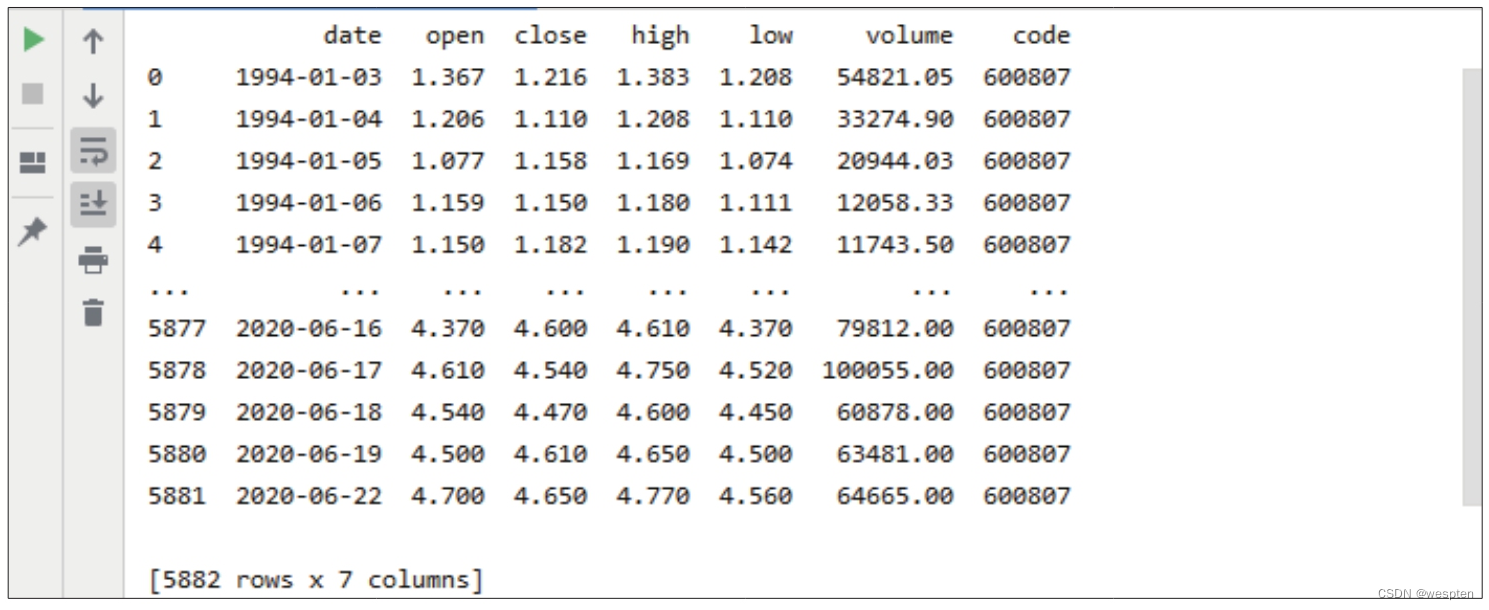``1   tianye.to_csv('tianye.csv')``

``1   Tianye = pd.read_csv('tianye.csv', index_col='date', parse_dates=['date'])　　# 将date列作为行标签，并将该列数据转换为date（日期）类型2   print(Tianye)``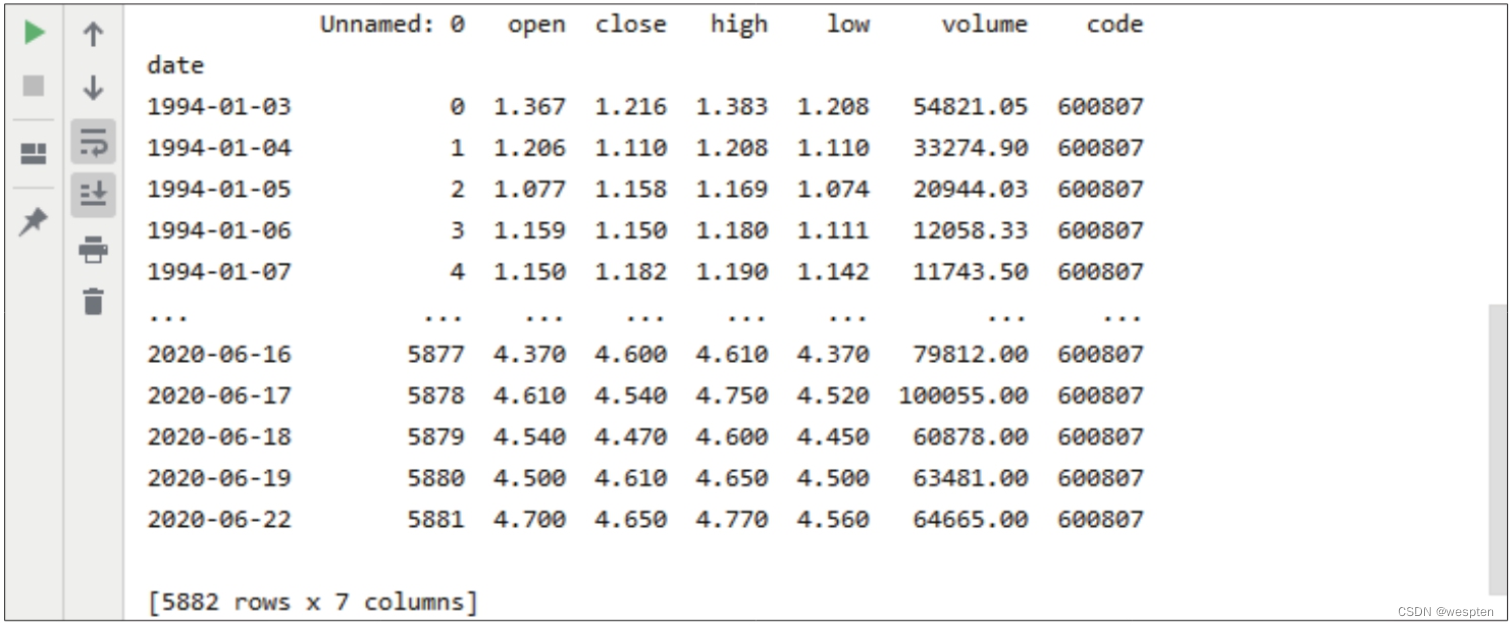``1   Tianye = Tianye['2010':'2019']  # 使用行标签做切片获取2010—2019年的数据``

``1   month_first = Tianye.resample('M').first()  # 获取每月第一天的股价数据2   month_first_money = month_first['open'].sum() * 1000　　# 计算每月第一天以开盘价买入1000股该股票的总支出3   month_max = Tianye.resample('M').last()  # 获取每月最后一天的股价数据4   month_max_money = month_max['close'].sum() * 1000  # 计算每月最后一天以收盘价卖出的总收入5   get_money = month_max_money - month_first_money  # 计算10年收益6   print(get_money)``

``1   本接口即将停止更新，请尽快使用Pro版接口：https://waditu.com/document/2``

Tushare Pro大幅提升了数据稳定性和获取速度，并将数据内容扩展到股票、基金、期货、债券、外汇、数字货币等领域，极大地减轻了金融分析人员在金融数据采集、加工、存储过程中的工作量。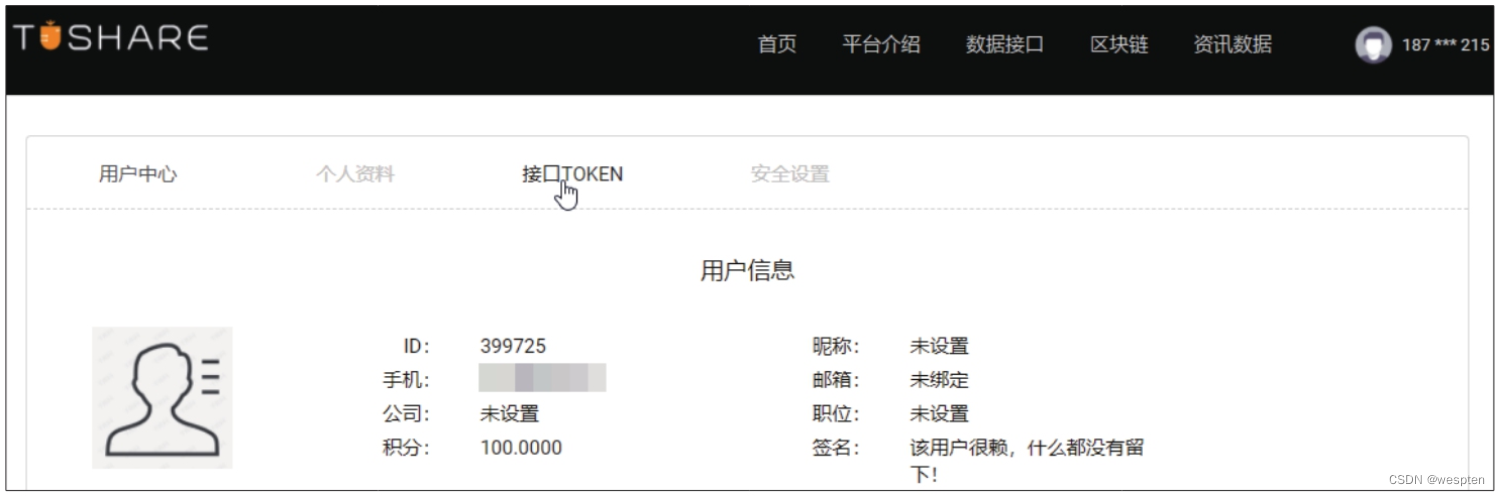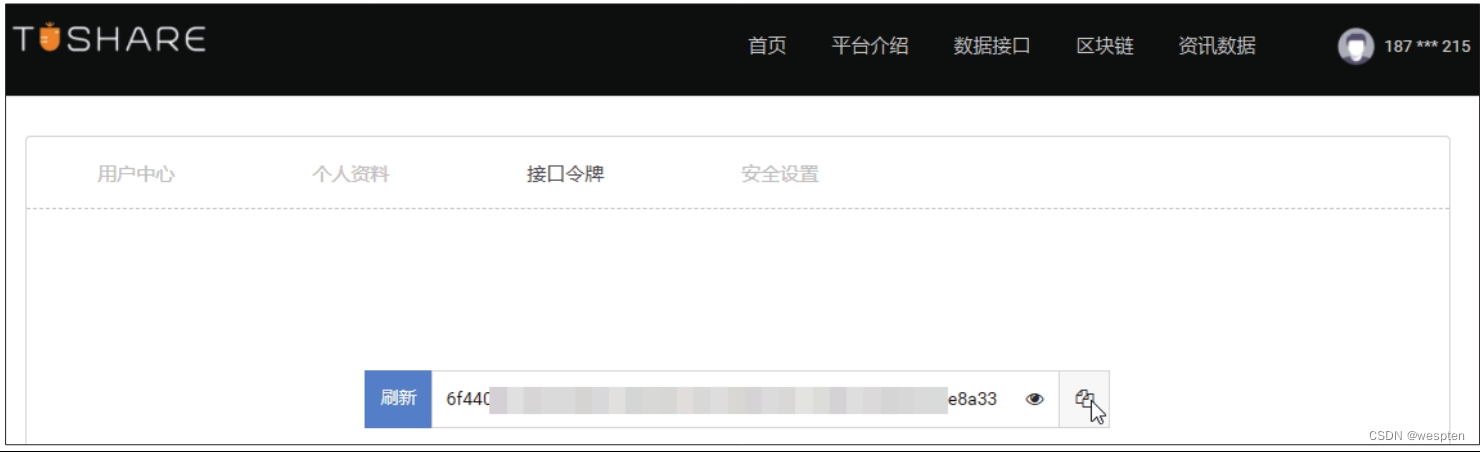``1   import tushare as ts2   ts.set_token('6f440************************************e8a33')3   pro = ts.pro_api()4   df = pro.daily(trade_date='20200918')5   print(df)``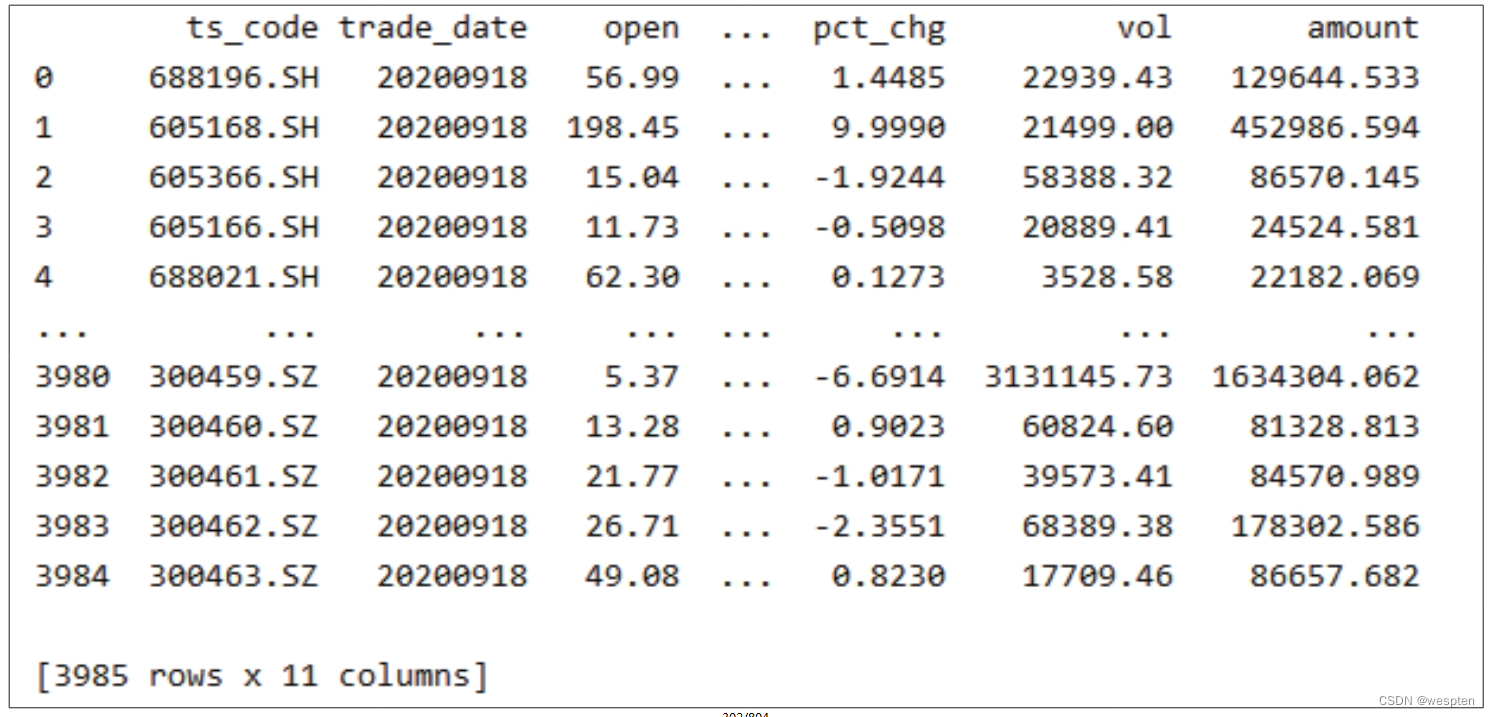## 四、Python数据分析实战

### 1、相关性分析

1. 获取股价数据

1）获取日K线级别的股价数据

``1   import tushare as ts2   data = ts.get_hist_data('000061', start='2018-01-01', end='2019-01-01')3   print(data.head(10))``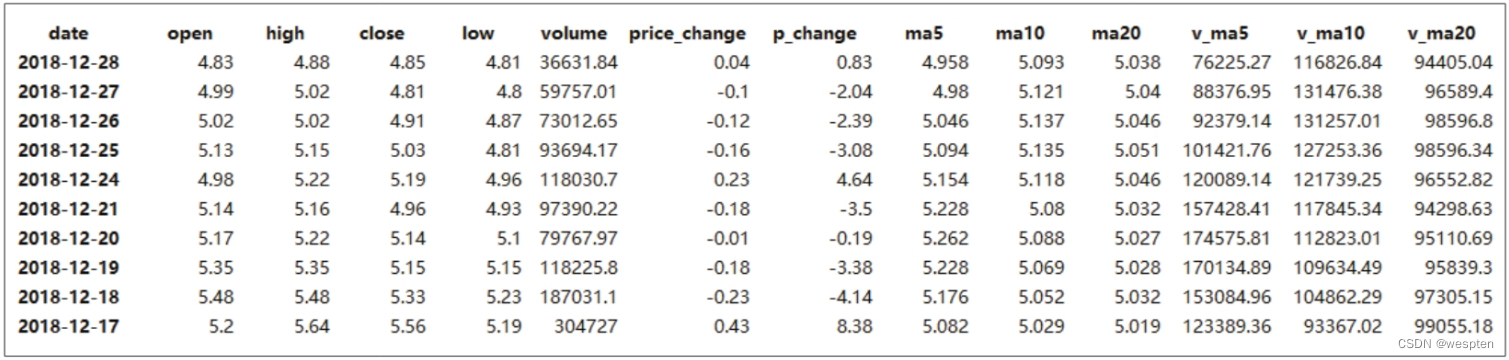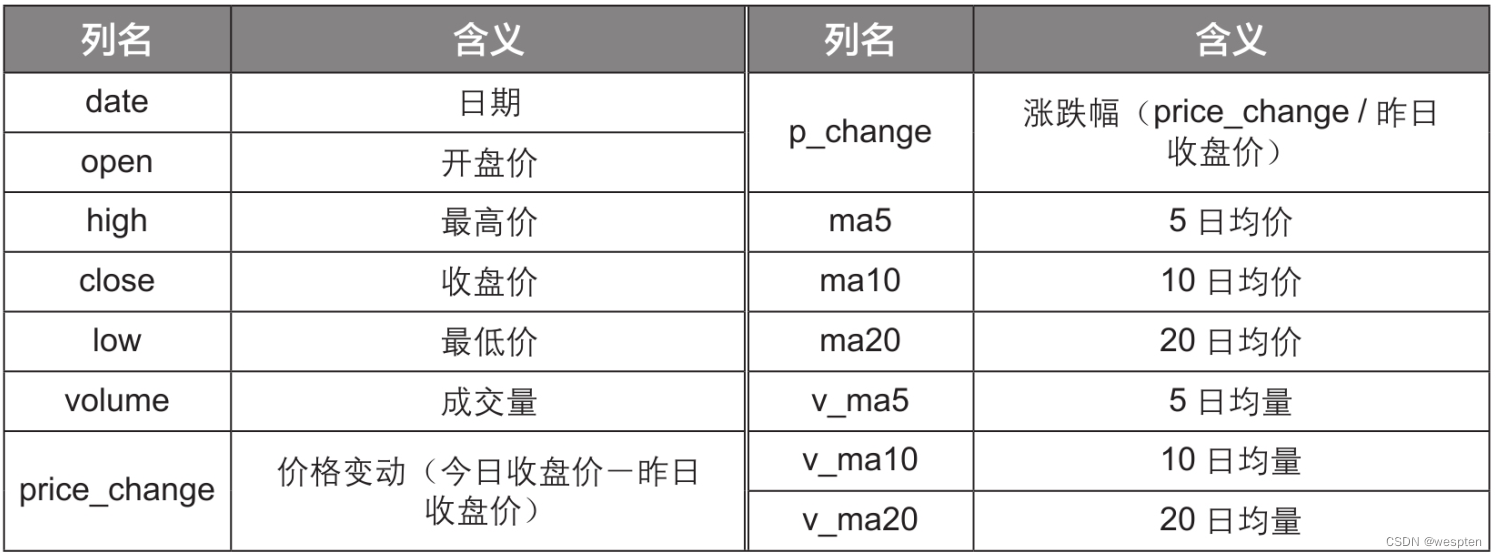``1   import pandas as pd2   pd.set_option('display.max_columns', None)``

2）获取分钟级别的股价数据

``1   data = ts.get_hist_data('000061', ktype='5')``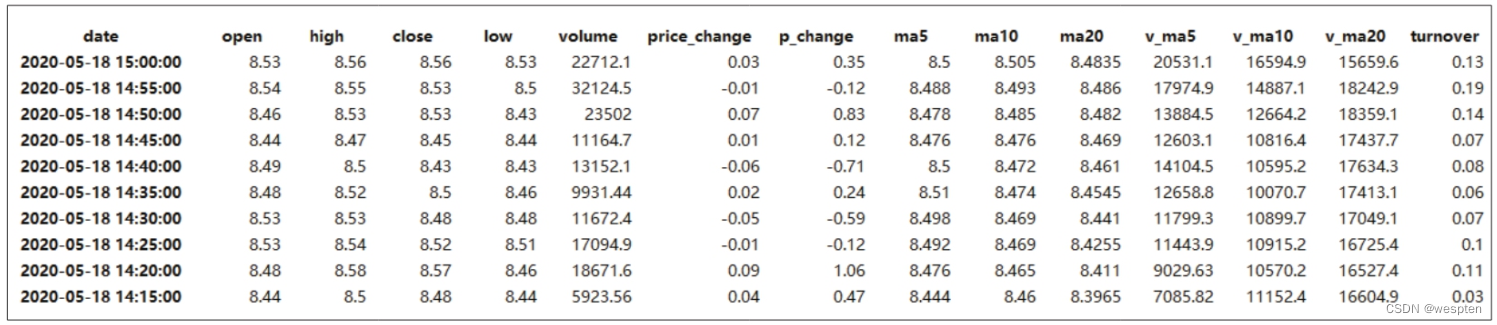3）获取实时股价数据

``1   data = ts.get_realtime_quotes('000061')``

``1   data = data[['code', 'name', 'price', 'bid', 'ask', 'volume', 'amount', 'date', 'time']]``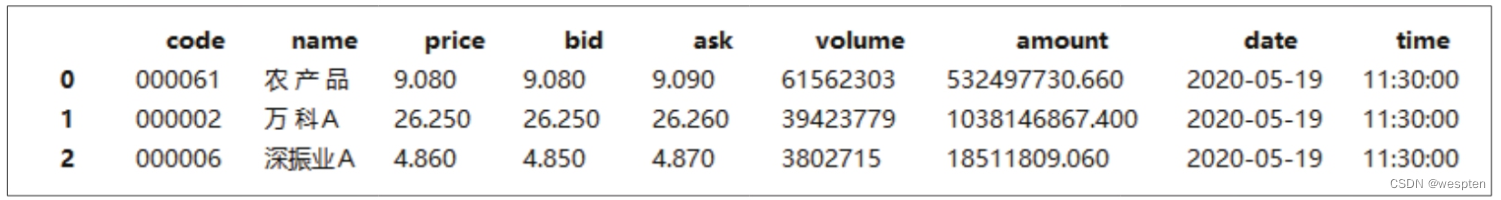4） 获取分笔股价数据

``1   data = ts.get_tick_data('000002', date='2018-12-12', src='tt')``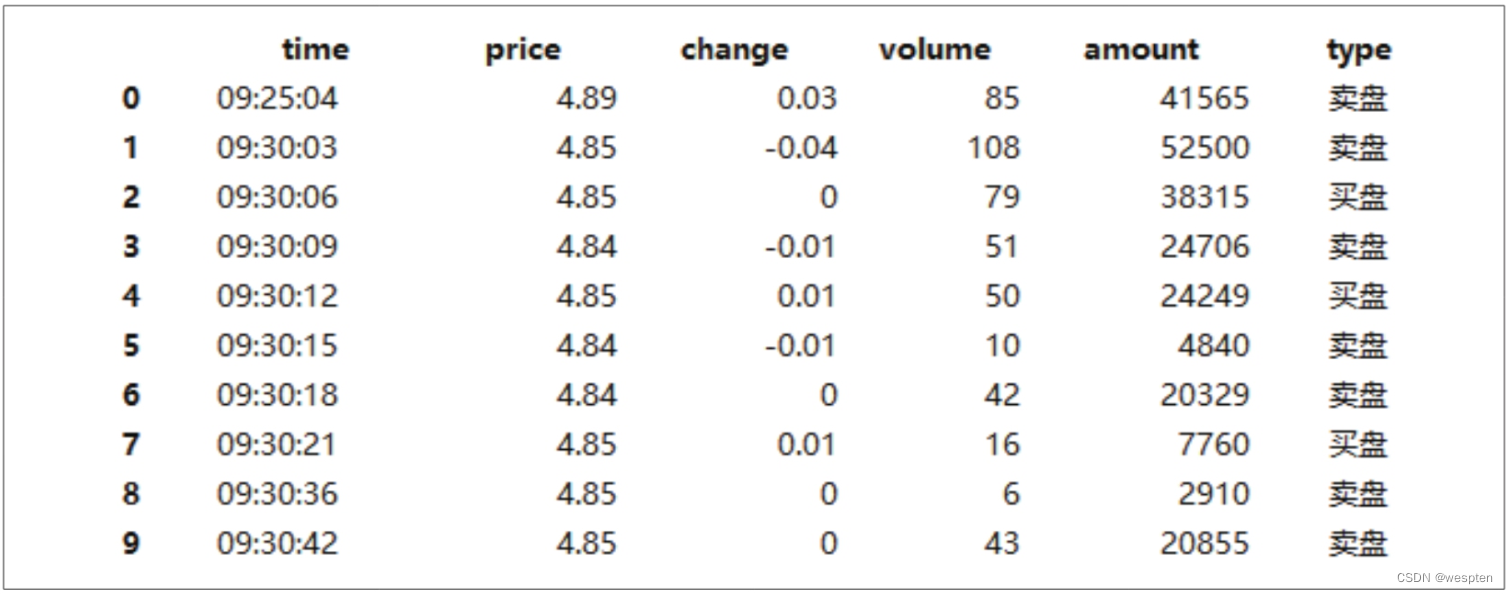5）获取指数信息

``1   data = ts.get_index()``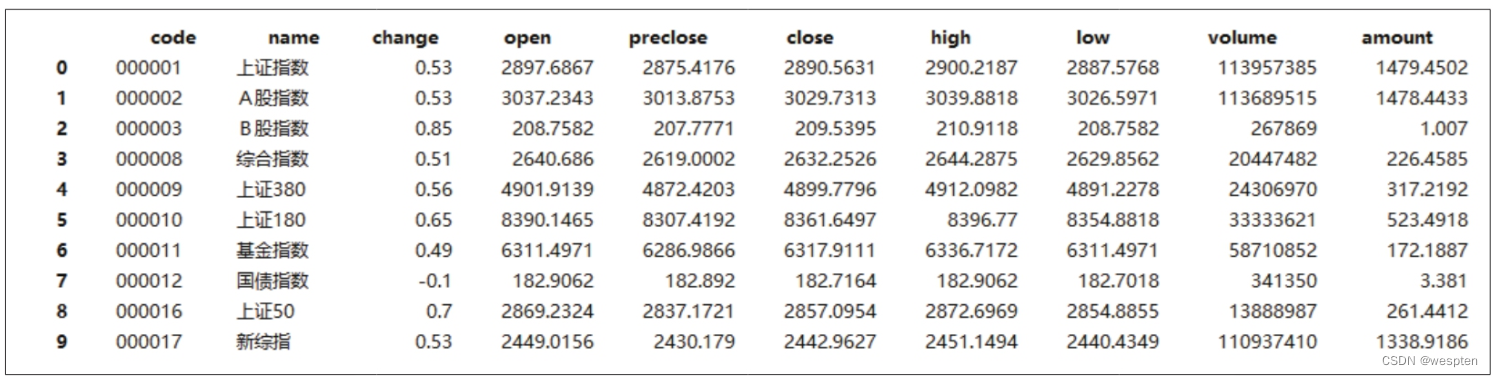2. 合并股价数据

``1   import tushare as ts2   data = ts.get_hist_data('000061', start='2018-01-01', end='2019-01-01')3   data.to_excel('农产品.xlsx')4   data1 = ts.get_hist_data('399300', start='2018-01-01', end='2019-01-01')5   data1.to_excel('沪深300.xlsx')``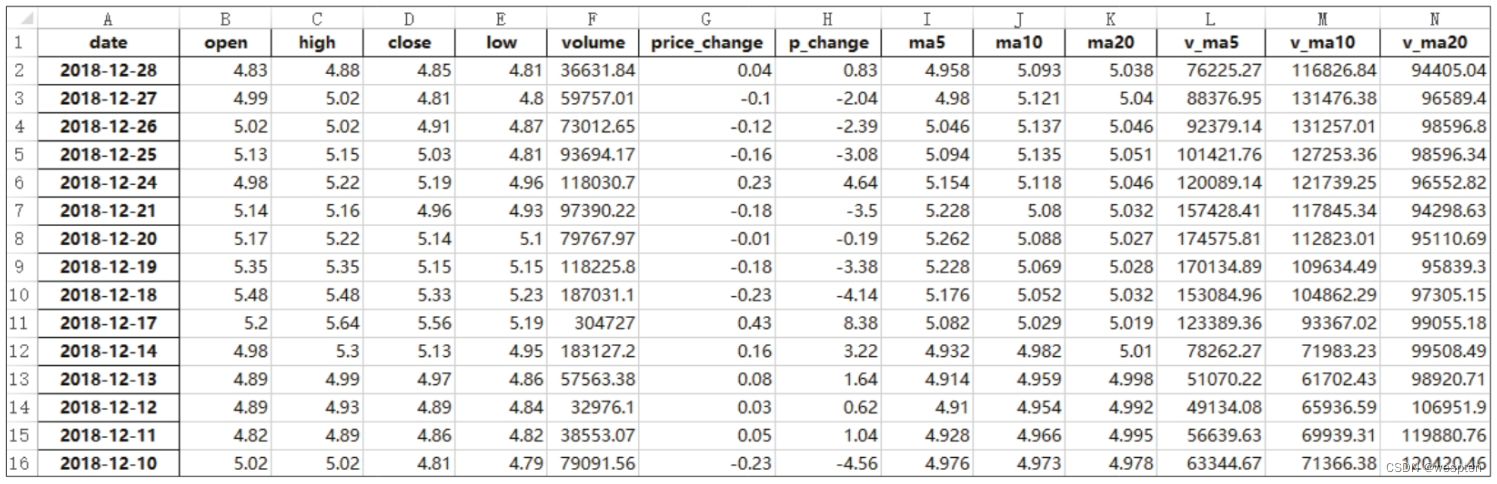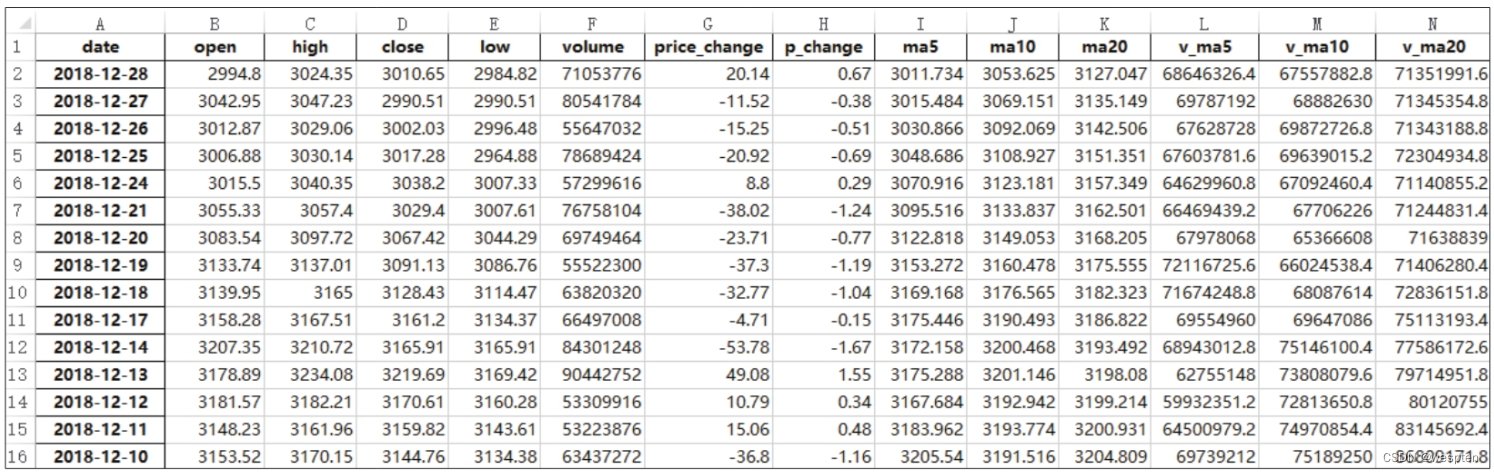``1   import pandas as pd2   stock = pd.read_excel('农产品.xlsx')3   stock = stock[['date', 'close']]4   stock = stock.rename(columns={'close': 'price_农产品'})5   stock1 = pd.read_excel('沪深300.xlsx')6   stock1 = stock1[['date', 'close']]7   stock1 = stock1.rename(columns={'close': 'price_沪深300'})8   data_merge = pd.merge(stock, stock1, on='date', how='inner')9   data_merge.to_excel('合并股价数据.xlsx', index=False)``3. 股价数据相关性分析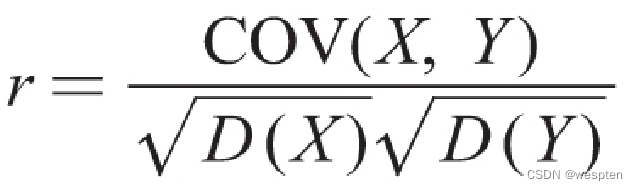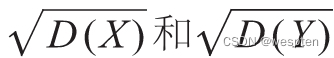r的取值范围为[-1，1]。r的正负表示相关性的类型：r为正值表示线性正相关，即两个变量的增长趋势相同；r为负值表示线性负相关，即两个变量的增长趋势相反；r为0表示不存在线性相关性。r的绝对值表示相关性的强弱，绝对值越接近1，说明相关性越强。

``1   from scipy.stats import pearsonr2   corr = pearsonr(X, Y)``

``1   import pandas as pd2   from scipy.stats import pearsonr3   data = pd.read_excel('合并股价数据.xlsx')4   corr = pearsonr(data['price_农产品'], data['price_沪深300'])5   print('相关系数r值为' + str(corr) + '，显著性水平P值为' + str(corr))``

``1   相关系数r值为0.9424989951941217，显著性水平P值为6.213473367651319e-112``

### 2、假设检验

t检验是一种推论统计量，用于确定在某些特征中两组的均值之间是否存在显著差异，主要用于数据集。t检验主要有两种类型：单样本t检验和双样本t检验。单样本t检验用于确定样本均值是否与已知或假设的总体均值具有统计学差异。双样本t检验用于比较两个独立组的均值，以确定是否有统计证据表明这两个独立组存在显著差异。

z检验用于在两组样本的总体方差未知时，检验两组数据表现情况的差异。它与t检验的区别在于：z检验常用于样本量较大的情况；而t检验则用于样本量较小、总体标准差未知的正态分布情况的数据。

F检验用于检验两个正态随机变量的总体方差是否相等，常用于判断应该选择使用t检验中的哪种检验方法，根据该检验方法计算出的方差比值可以用来检验两组数据是否存在显著性差异。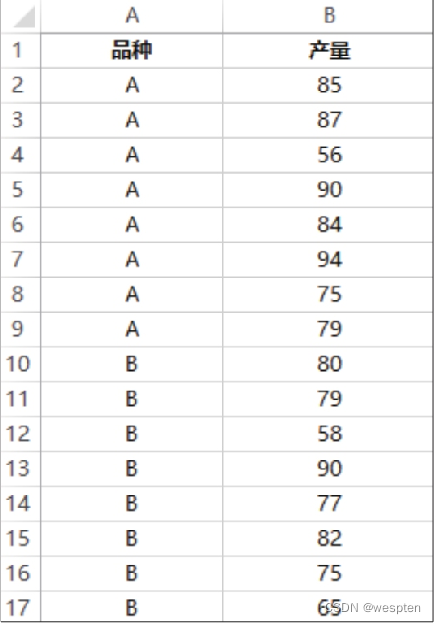``1   import pandas as pd2   data = pd.read_excel('样本数据.xlsx')3   print(data.head())``

``1         品种   产量2   0      A     853   1      A     874   2      A     565   3      A     906   4      A     84``

``1   x = data[data['品种'] == 'A']['产量']2   y = data[data['品种'] == 'B']['产量']``

``1   from scipy.stats import ttest_ind2   print(ttest_ind(x, y))``

``1   Ttest_indResult(statistic=1.0044570121313174, pvalue=0.3322044373983689)``

### 3、描述性统计分析

1. 描述性统计指标的计算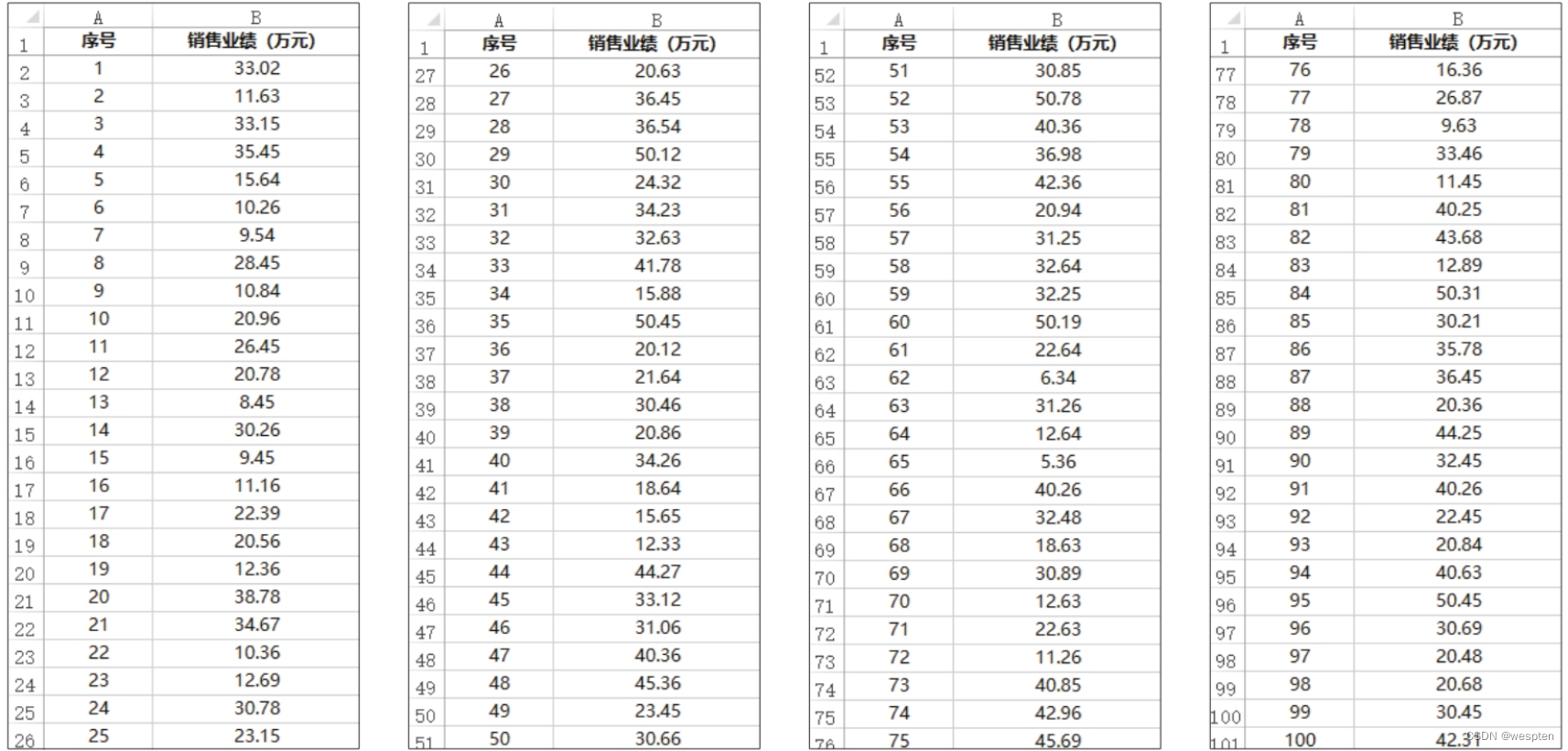``1   import pandas as pd2   data = pd.read_excel('销售业绩统计.xlsx', index_col='序号')3   print(data.head())``

``1        销售业绩（万元）2   序号3   1      33.024   2      11.635   3      33.156   4      35.457   5      15.64``

SciPy是一个基于NumPy模块开发的高级模块，它提供了许多用于解决科学计算问题的数学算法和函数，而且在符号计算、信号处理、数值优化等方面有突出表现。SciPy模块包含很多子模块，其中，子模块stats在统计分布分析方面有很大的作用。

``1   from numpy import mean, median2   from scipy.stats import mode3   mean = mean(data['销售业绩（万元）'])4   median = median(data['销售业绩（万元）'])5   mode = mode(data['销售业绩（万元）'])6   print('均值：' + str(mean))7   print('中位数：' + str(median))8   print('众数：' + str(mode))``

``1   均值：27.9525999999999932   中位数：30.4553   众数：36.45``

``1   from numpy import ptp, var, std2   ptp = ptp(data['销售业绩（万元）'])3   var = var(data['销售业绩（万元）'])4   std = std(data['销售业绩（万元）'])5   print('极差：' + str(ptp))6   print('方差：' + str(var))7   print('标准差：' + str(std))``

``1   极差：45.422   方差：146.678775240000023   标准差：12.1``

2. 数据的分布状态分析

``1   import numpy as np2   standard_normal = pd.Series(np.random.normal(0, 1, size=1000000))3   normal_p = standard_normal.skew()4   normal_f = standard_normal.kurt()5   print('标准正态分布的偏度：' + str(normal_p))6   print('标准正态分布的峰度：' + str(normal_f))``

``1   标准正态分布的偏度：-0.0007279618700804892   标准正态分布的峰度：0.001021764823831539``

``1   p = data['销售业绩（万元）'].skew()2   f = data['销售业绩（万元）'].kurt()3   print('偏度：' + str(p))4   print('峰度：' + str(f))``

``1   偏度：0.032795718478277272   峰度：-0.9749688324183667``

``1   import seaborn as sns2   import matplotlib.pyplot as plt3   plt.rcParams['font.sans-serif'] = ['SimHei']4   plt.rcParams['axes.unicode_minus'] = False5   sns.kdeplot(standard_normal, shade=True, label='标准正态分布')6   sns.kdeplot(data['销售业绩（万元）'], shade=True, label='销售业绩分布')7   plt.show()``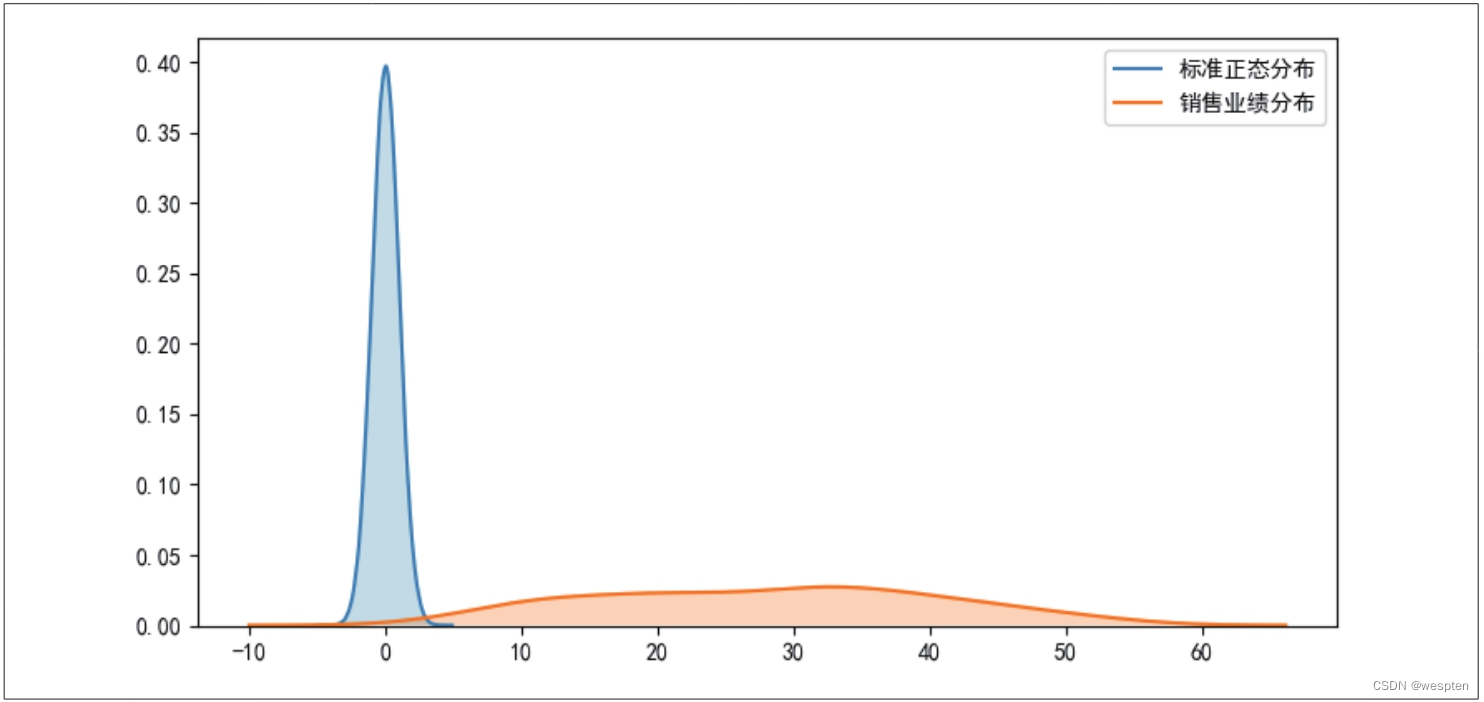3. 数据的频数和频率分析

``1   frequency = data['销售业绩（万元）'].value_counts()2   percentage = frequency / len(data['销售业绩（万元）'])3   print(frequency.head())4   print(percentage.head())``

``1   50.45      22   36.45      23   40.36      24   40.26      25   22.63      16   Name: 销售业绩（万元）, dtype: int647   50.45      0.028   36.45      0.029   40.36      0.0210   40.26      0.0211   22.63      0.0112   Name: 销售业绩（万元）, dtype: float64``

``1   import matplotlib.pyplot as plt2   plt.rcParams['font.sans-serif'] = ['SimHei']3   plt.rcParams['axes.unicode_minus'] = False4   plt.hist(data['销售业绩（万元）'], bins=9, density=False, color='g', edgecolor='k', alpha=0.75)5   plt.xlabel('销售业绩')6   plt.ylabel('频数')7   plt.title('销售业绩频数分布直方图')8   plt.show()``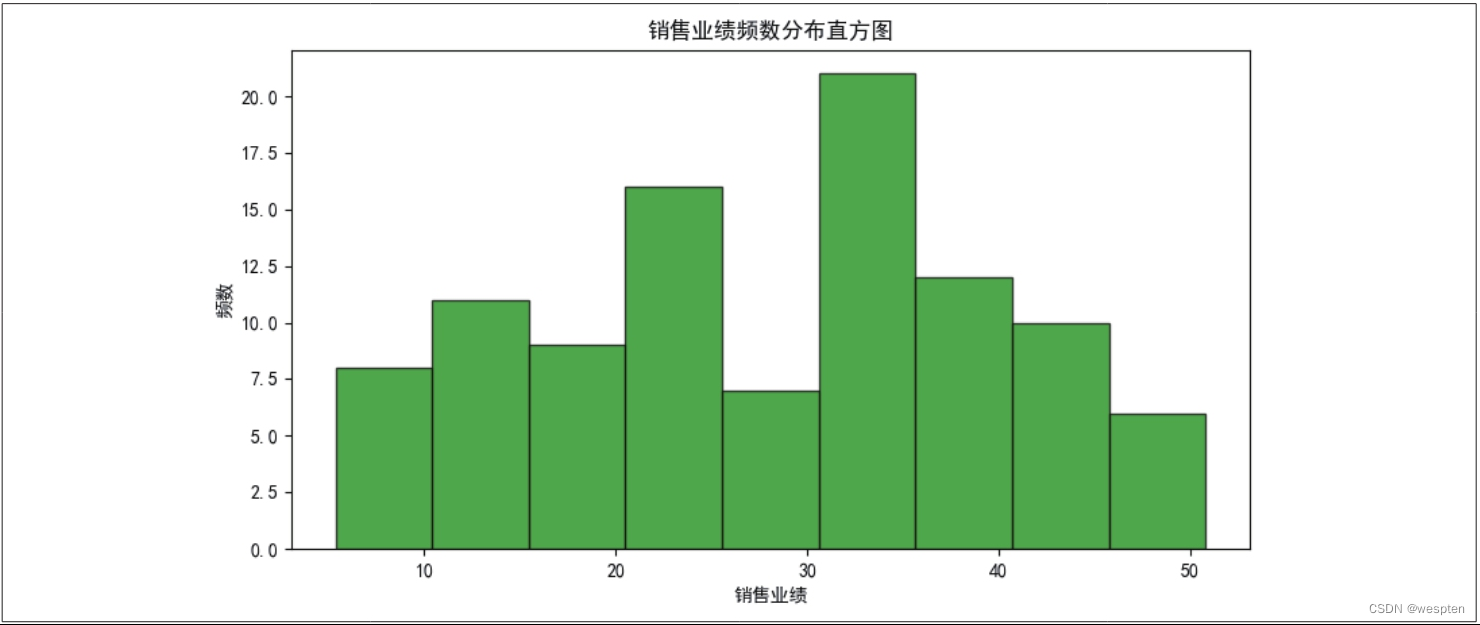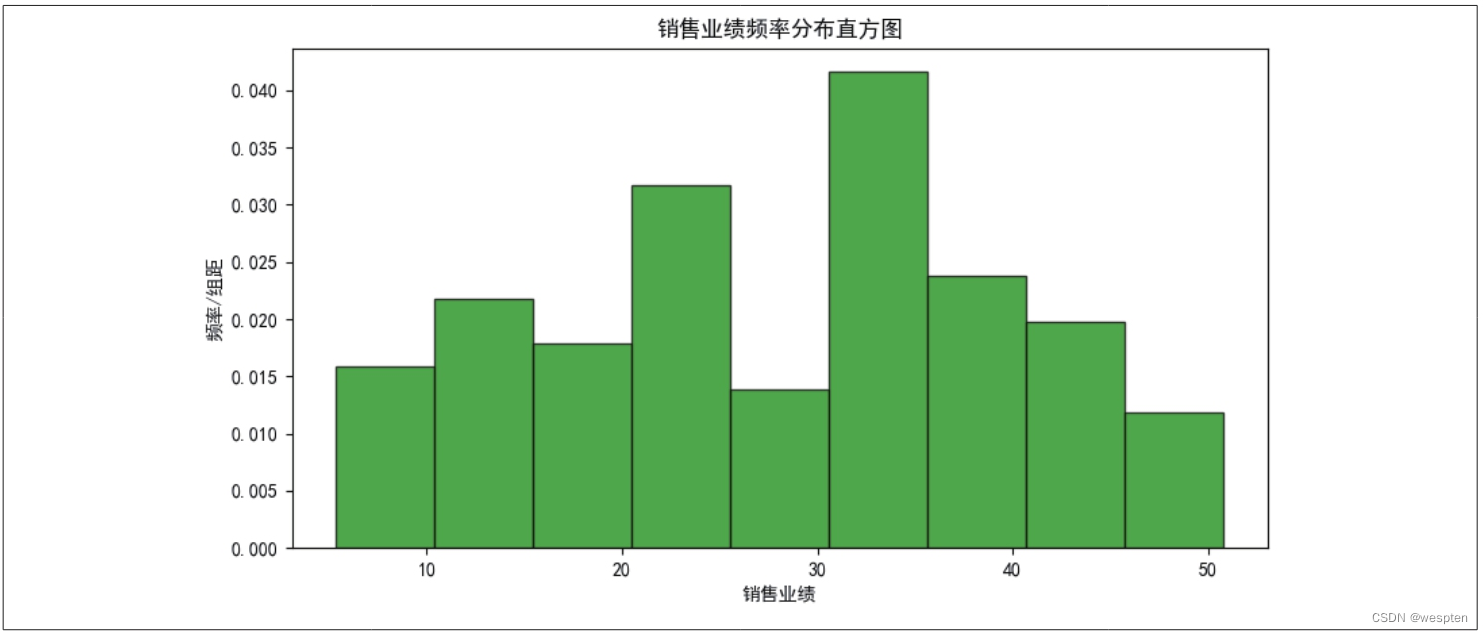hist()函数的参数bins的值设置为一个整型数字时表示自动均匀分组，也可以自行设定分组组距，如设置bins=[5, 10, 15, 20, 25, 30, 35, 40, 45, 50, 55]。